# RD Sharma Solutions For Class 7 Maths Chapter - 2 Fractions

RD Sharma Solutions for Class 7 Chapter 2 Fractions PDF are available here. Students can download pdf of the solutions from the links given below. Class 7 is a stage where the students are introduced to several new topics. Our experts formulate those topics to help students in their exam preparation and achieve excellent marks in Maths. The solutions are stepwise and detailed to make learning easy. Students who wish to excel in exams must practise using RD Sharma Solutions for Class 7. This solution guide also builds a foundation about the basic concepts and real life applications in the student’s life. The solutions to all questions in RD Sharma books are given here in a detailed and step by step way to help the students understand the topics in a more effective way. In this chapter, we will learn about the comparison of fractions, fundamental operations on fractions, equivalent fractions and so on.

## Download the pdf of RD Sharma For Class 7 Maths Chapter 2 Fractions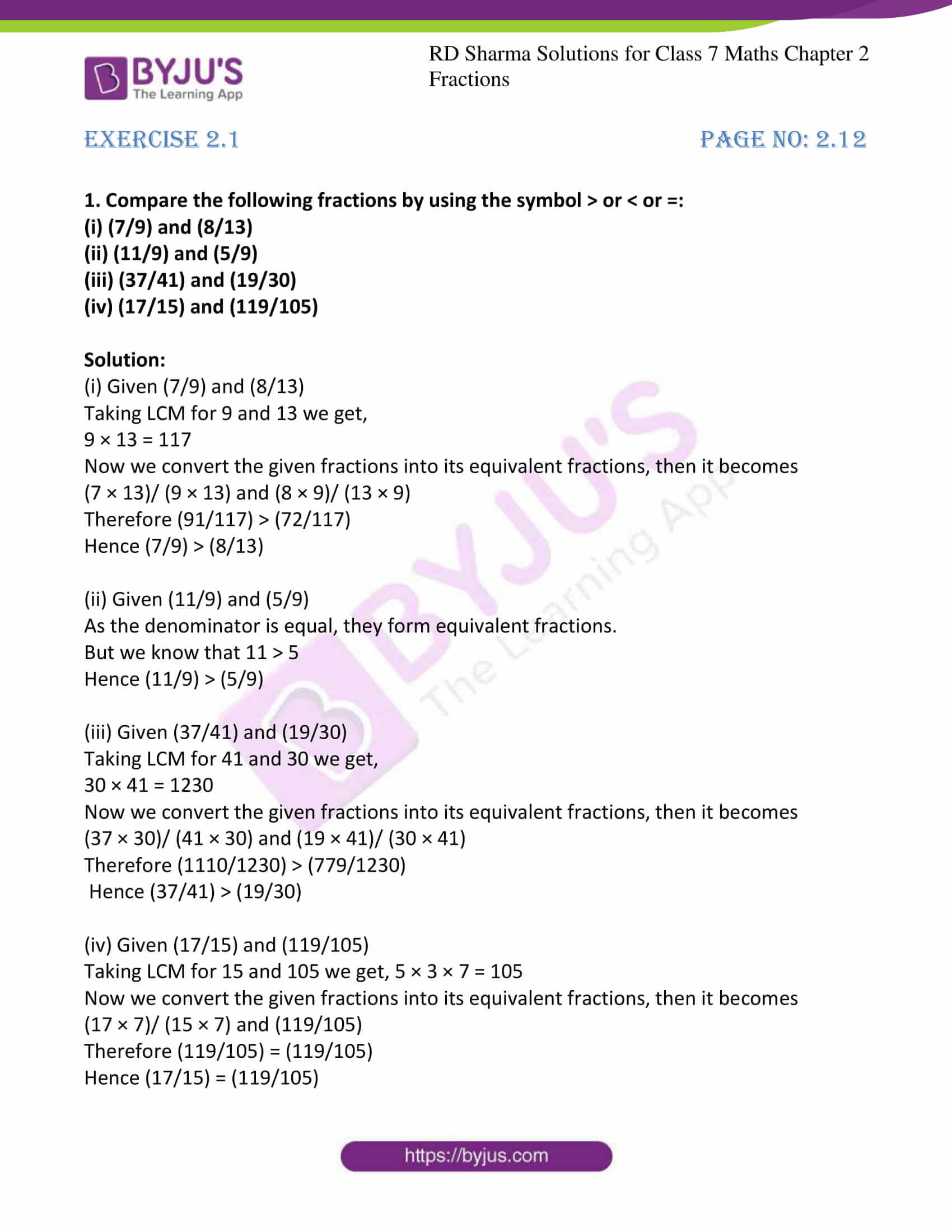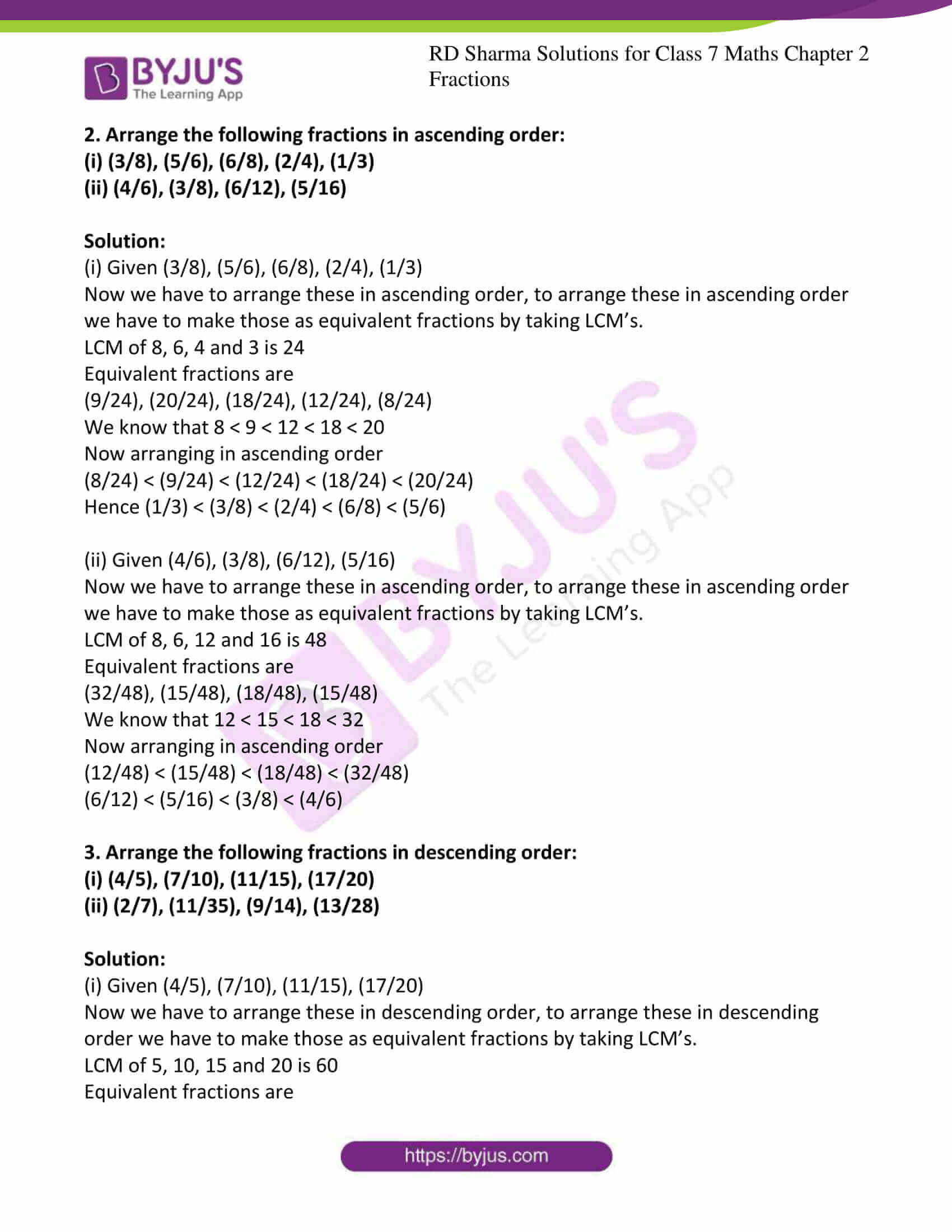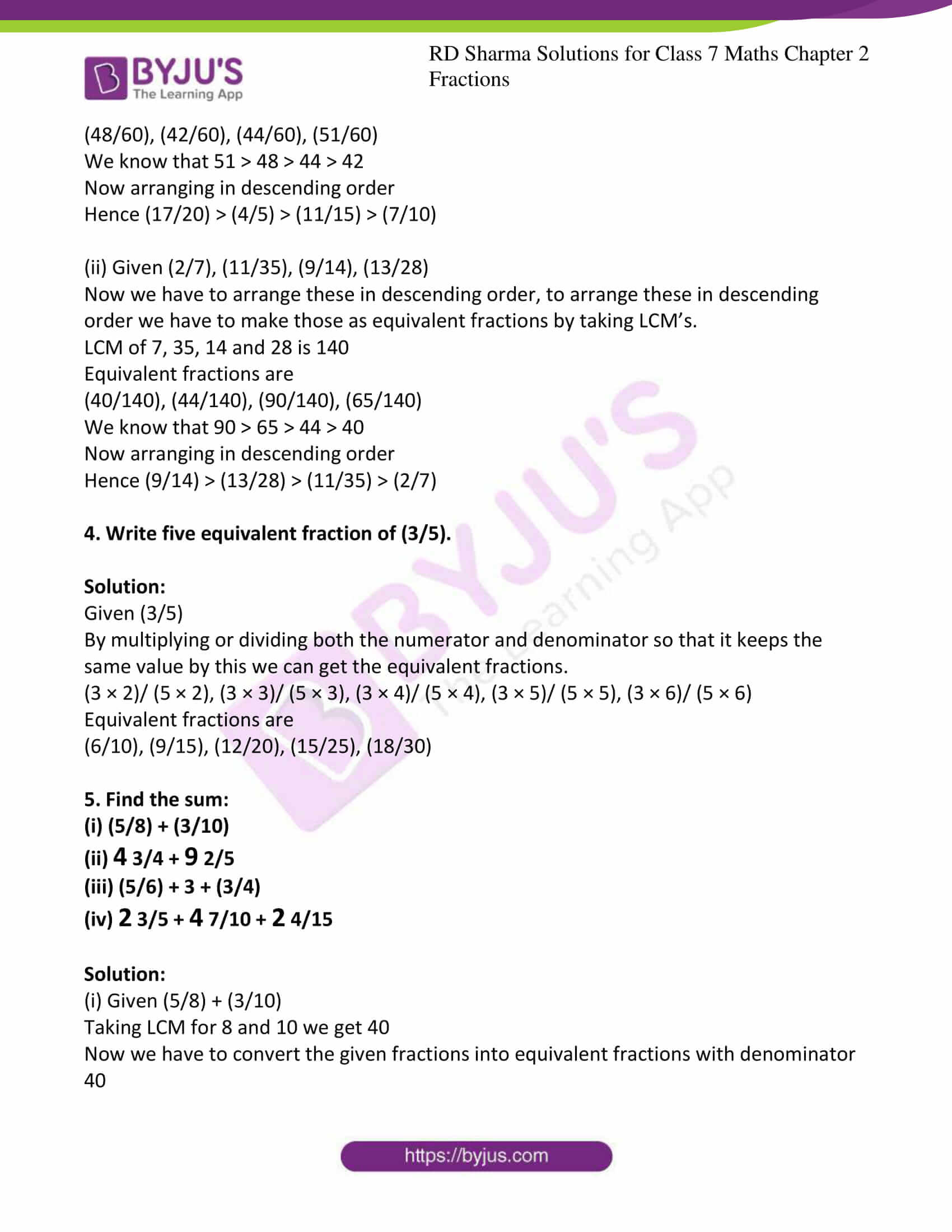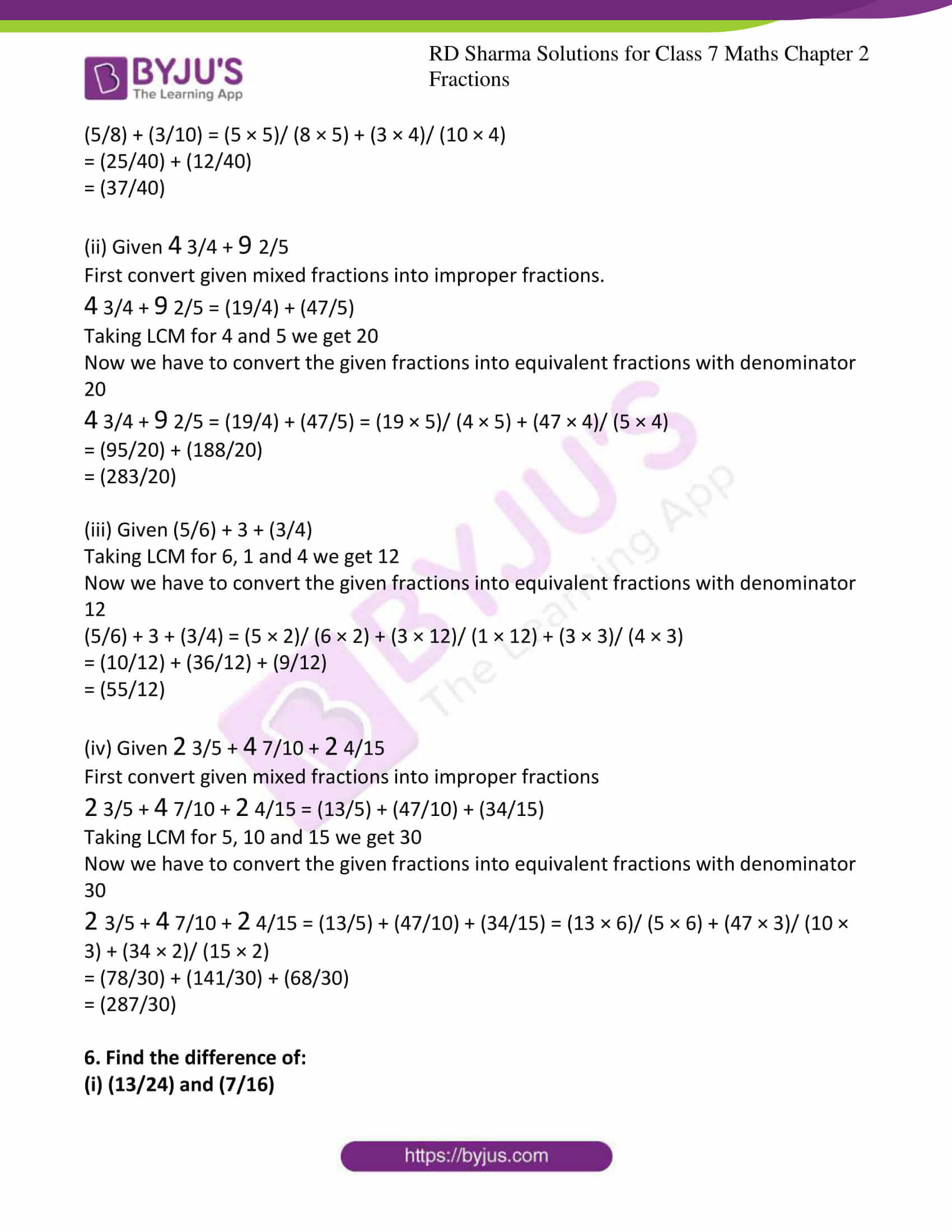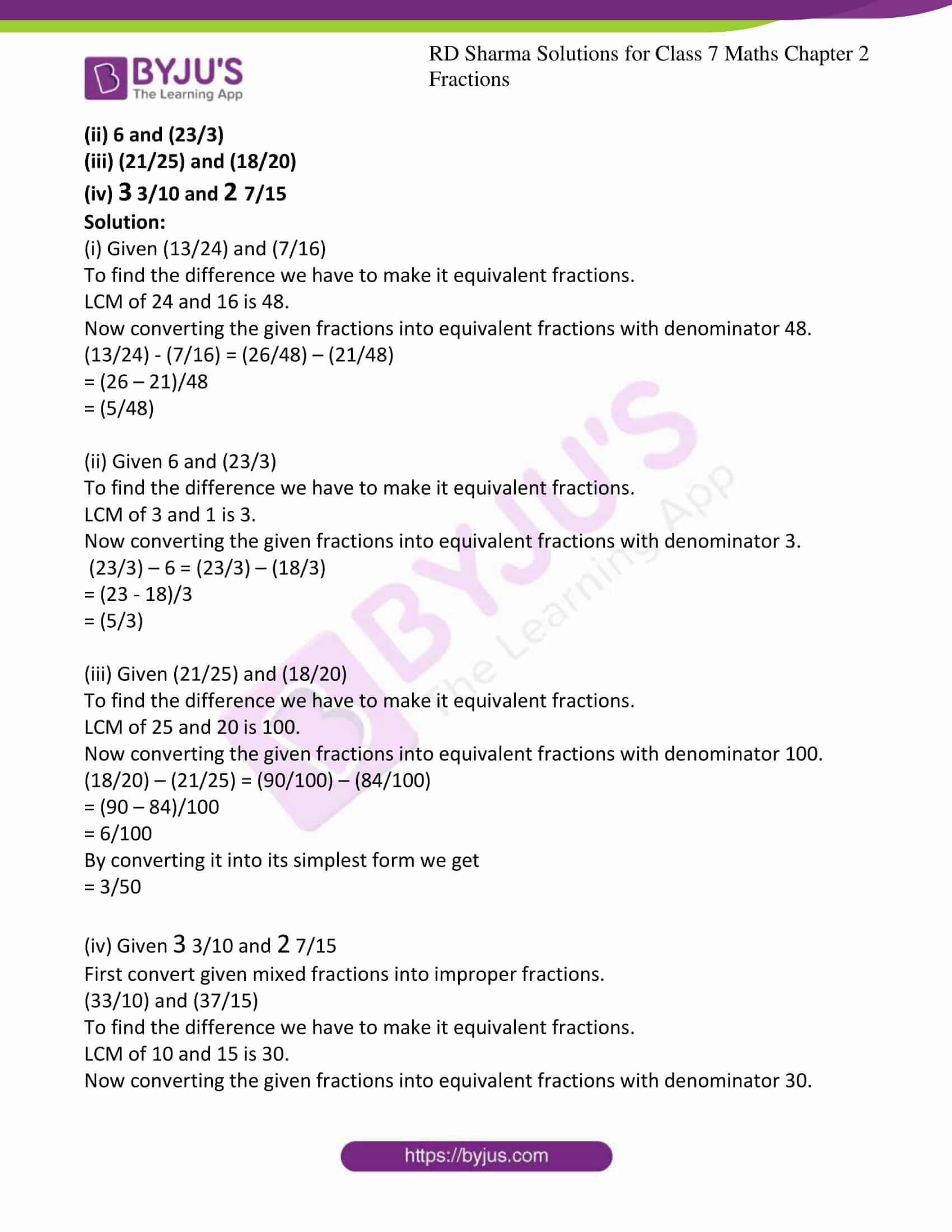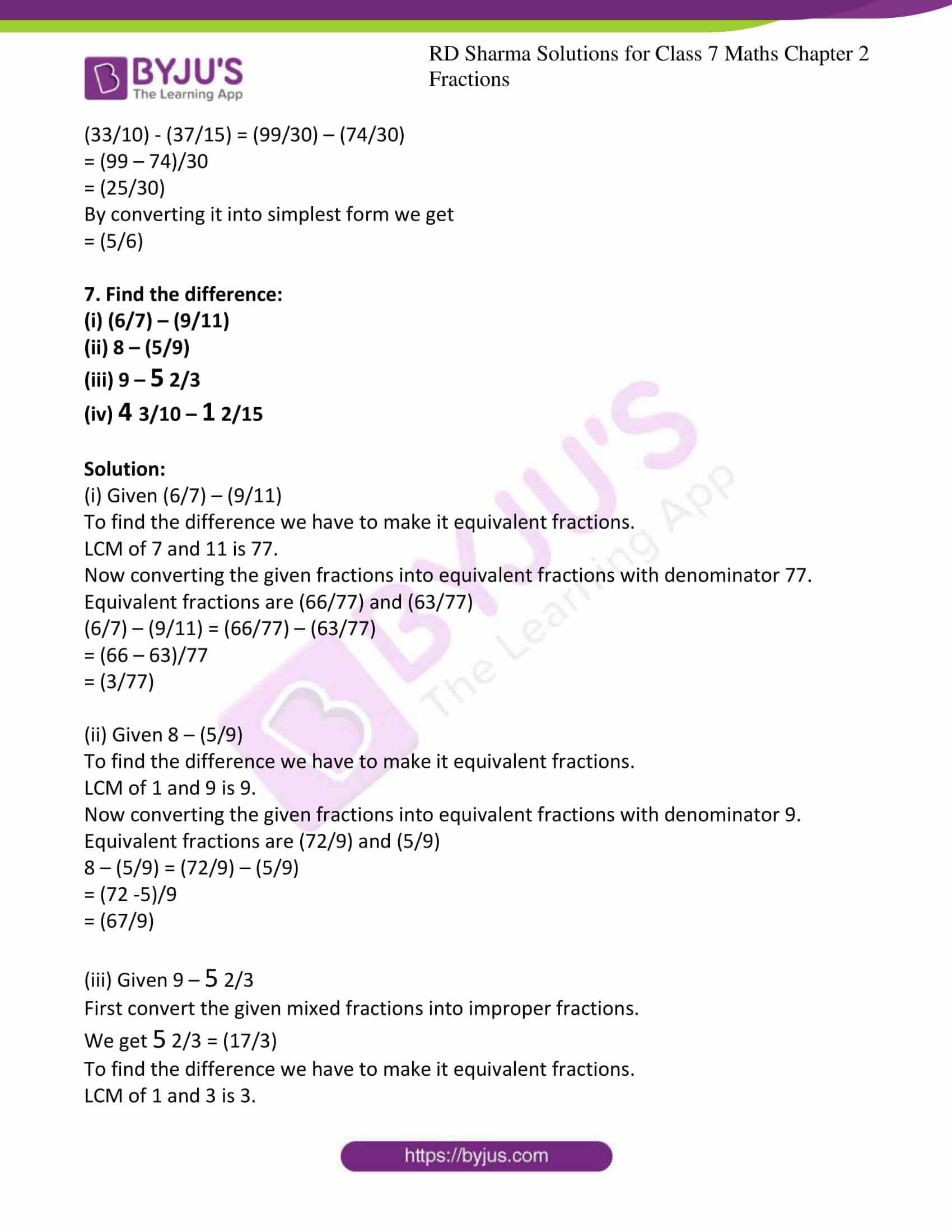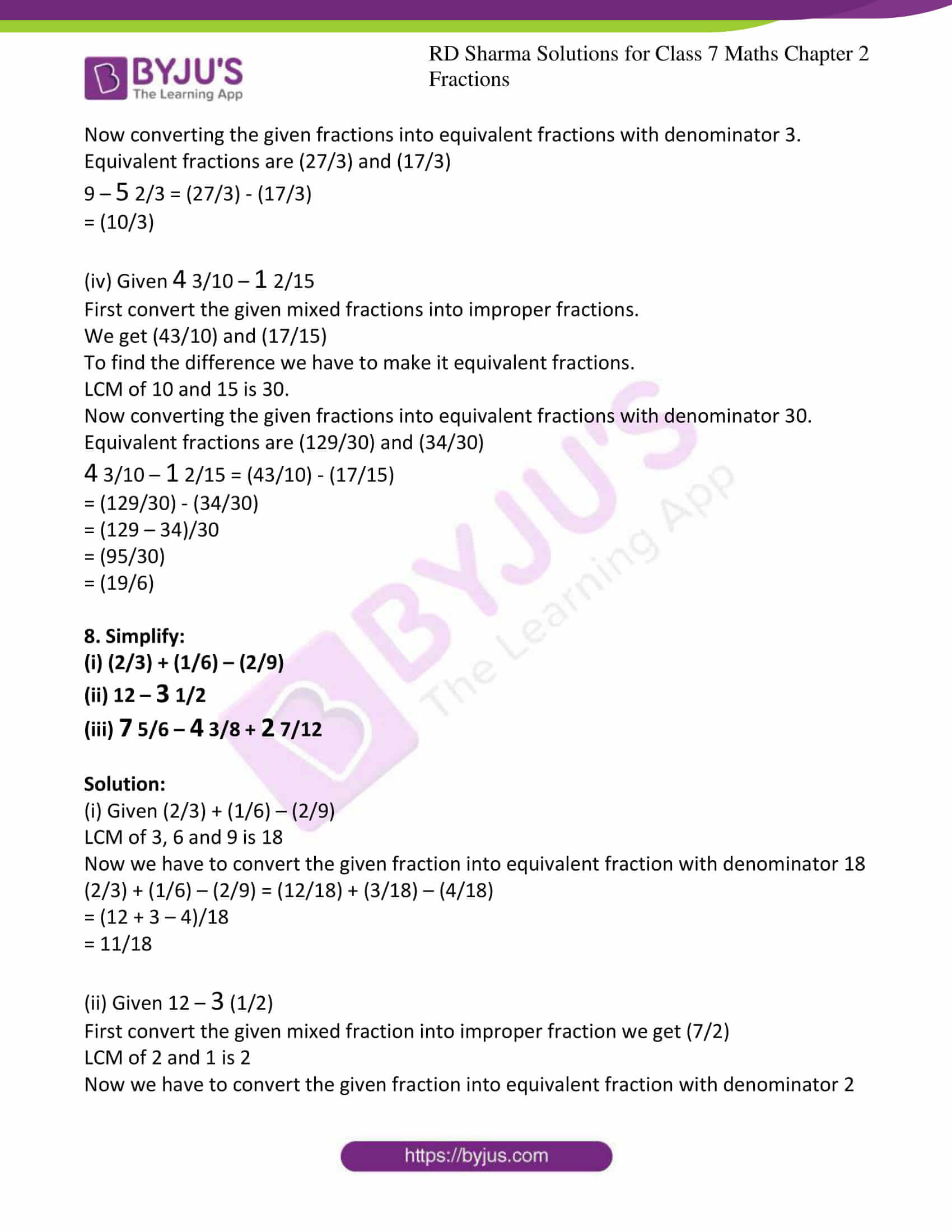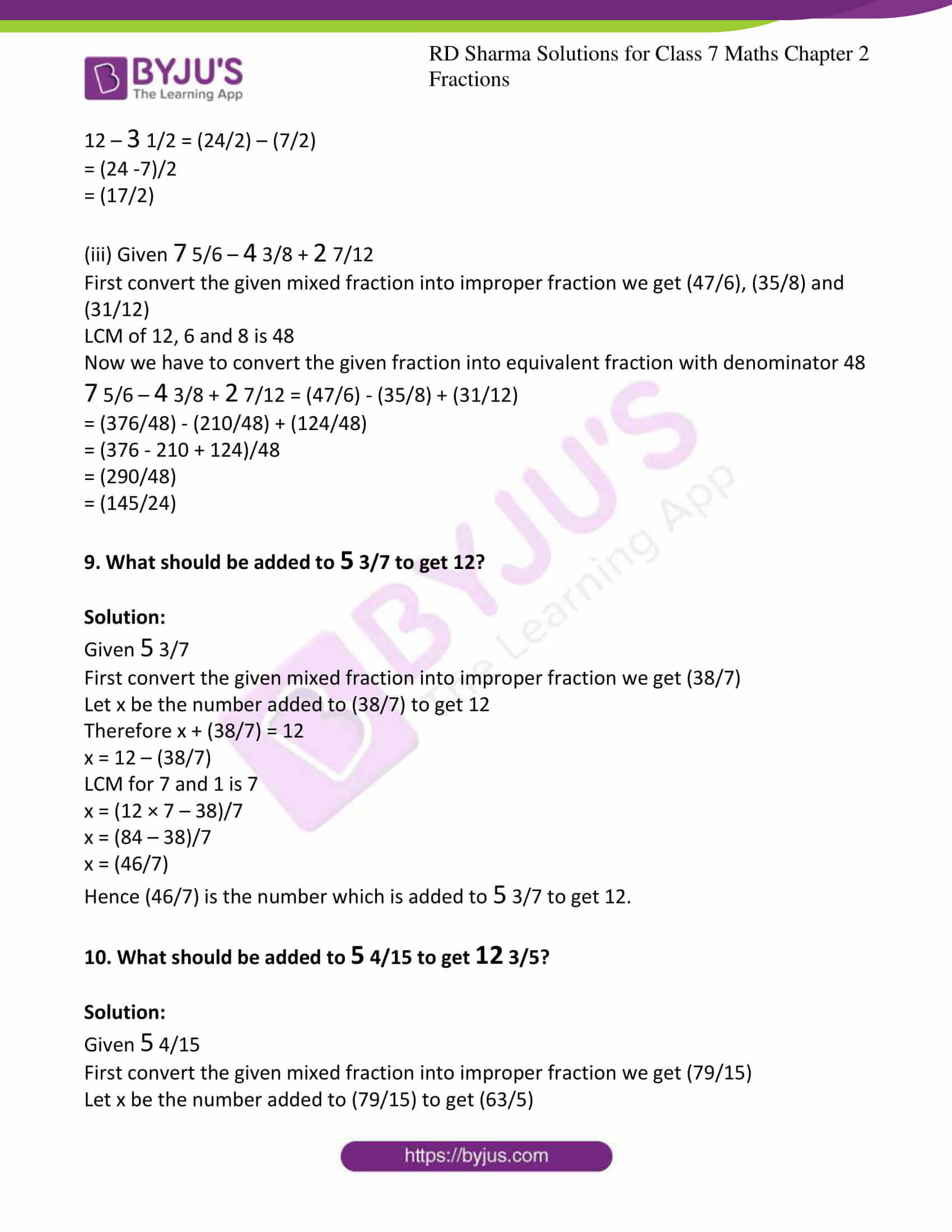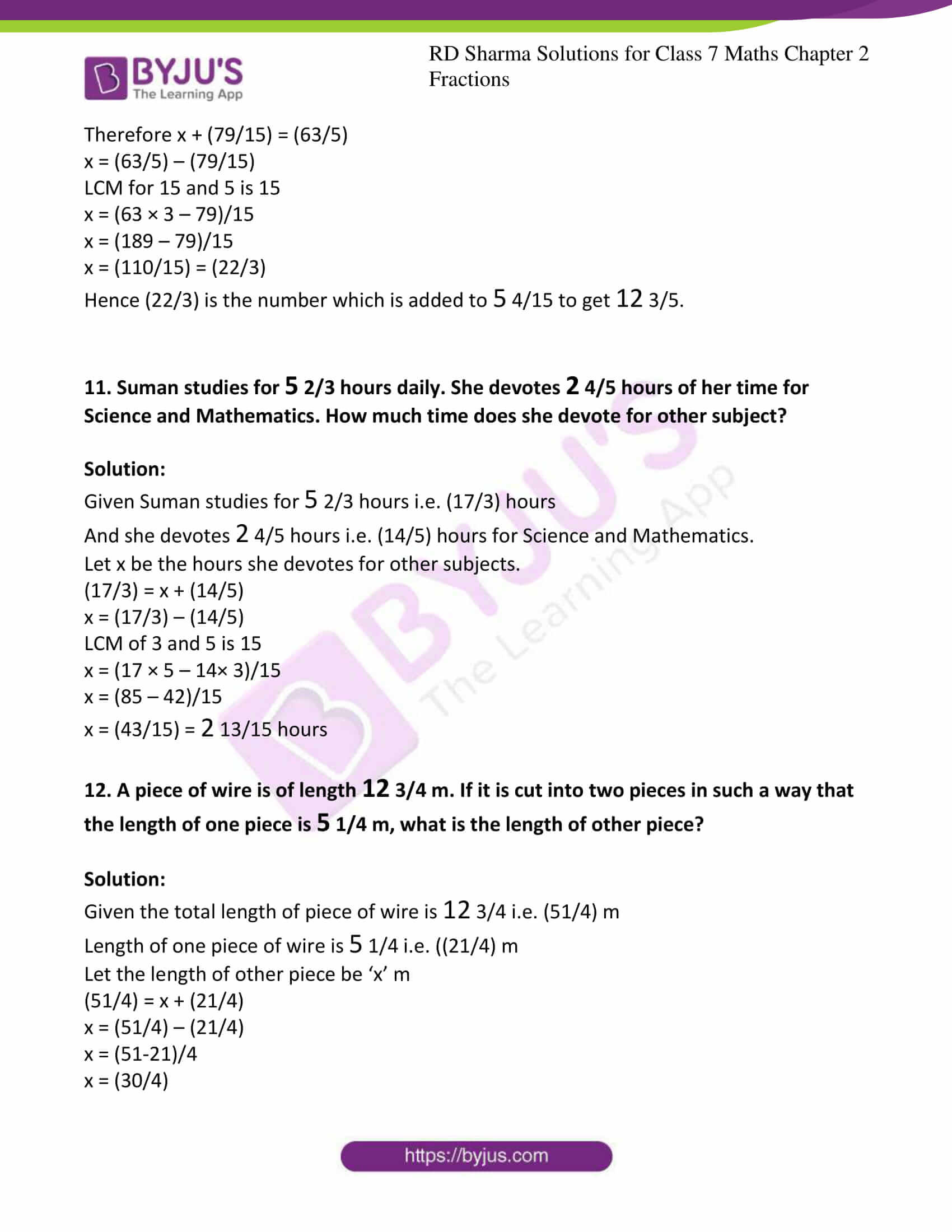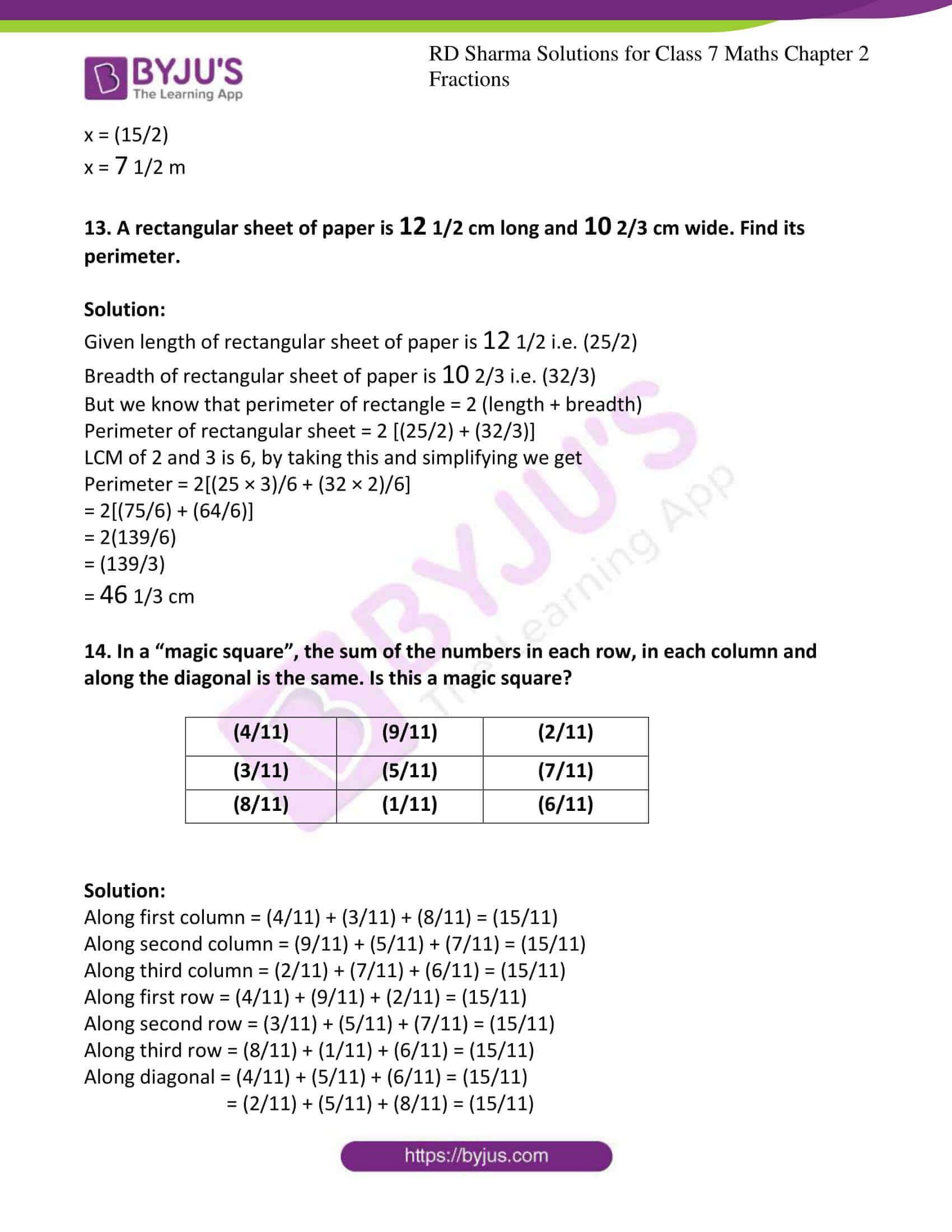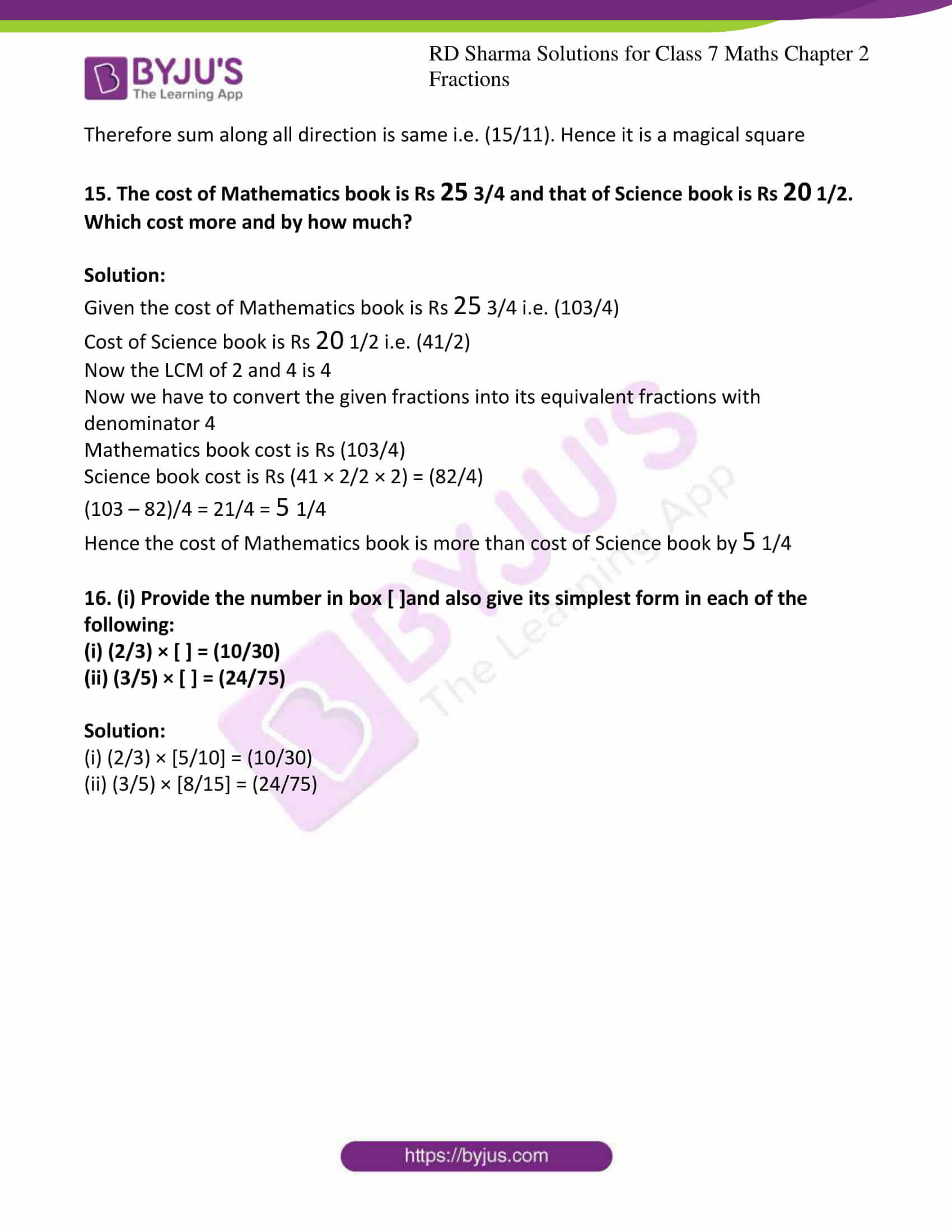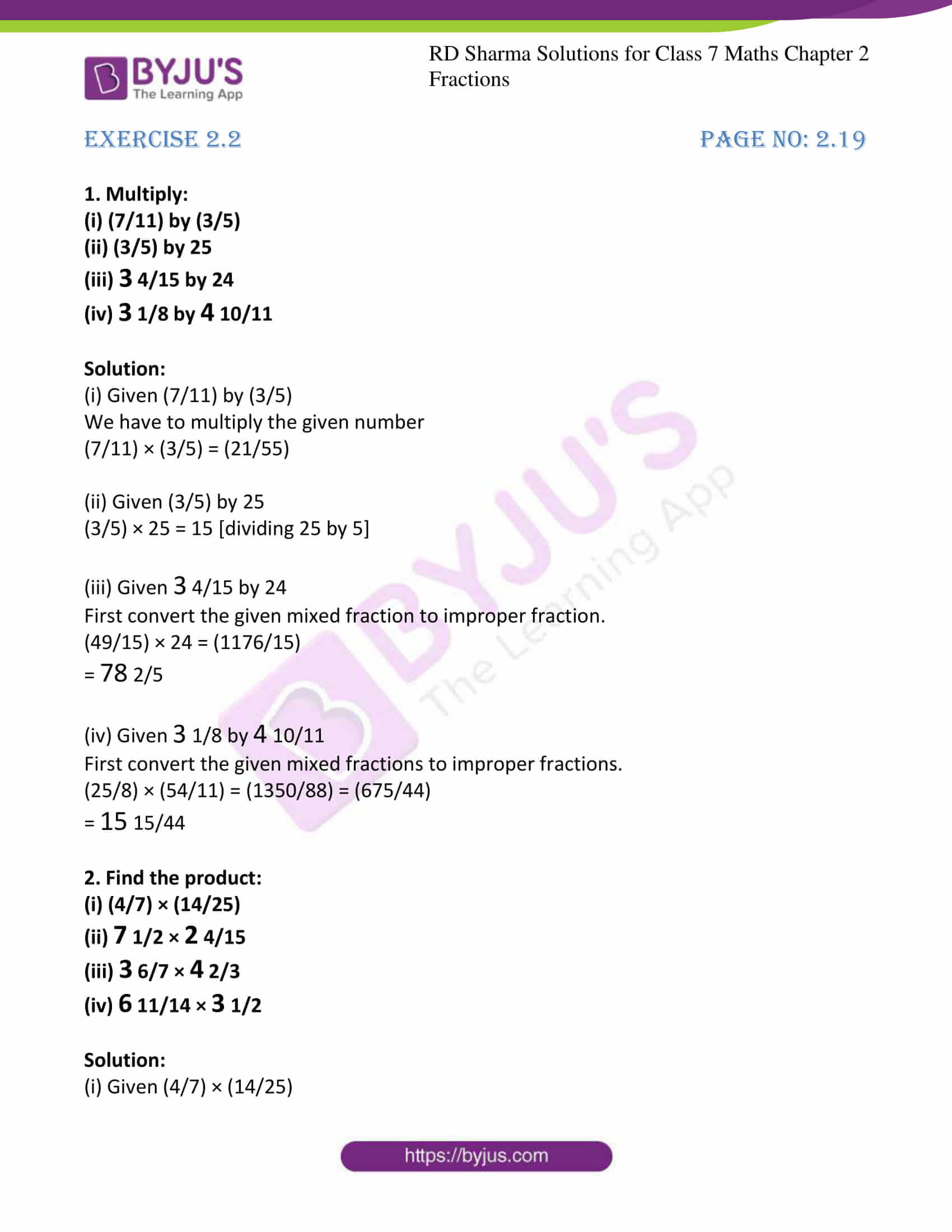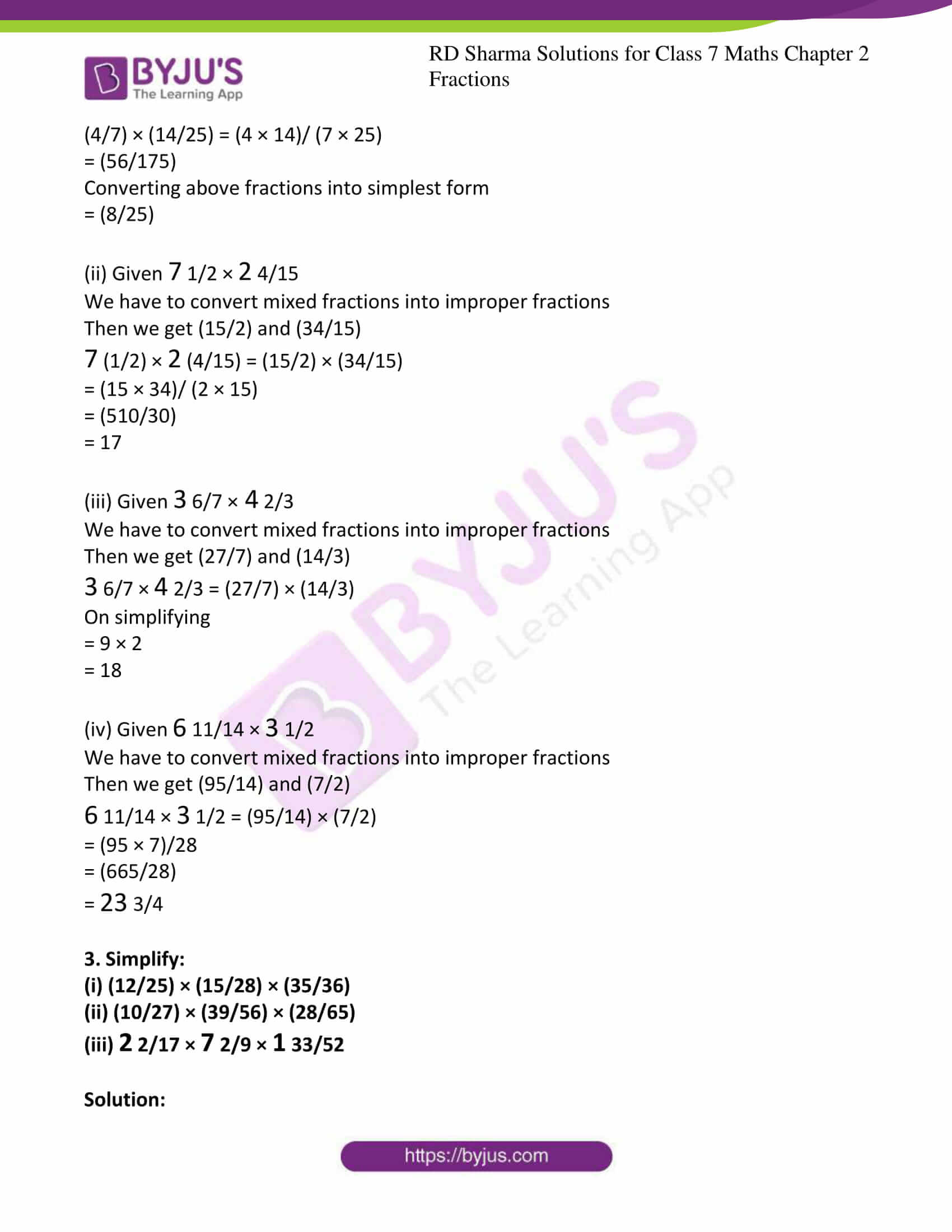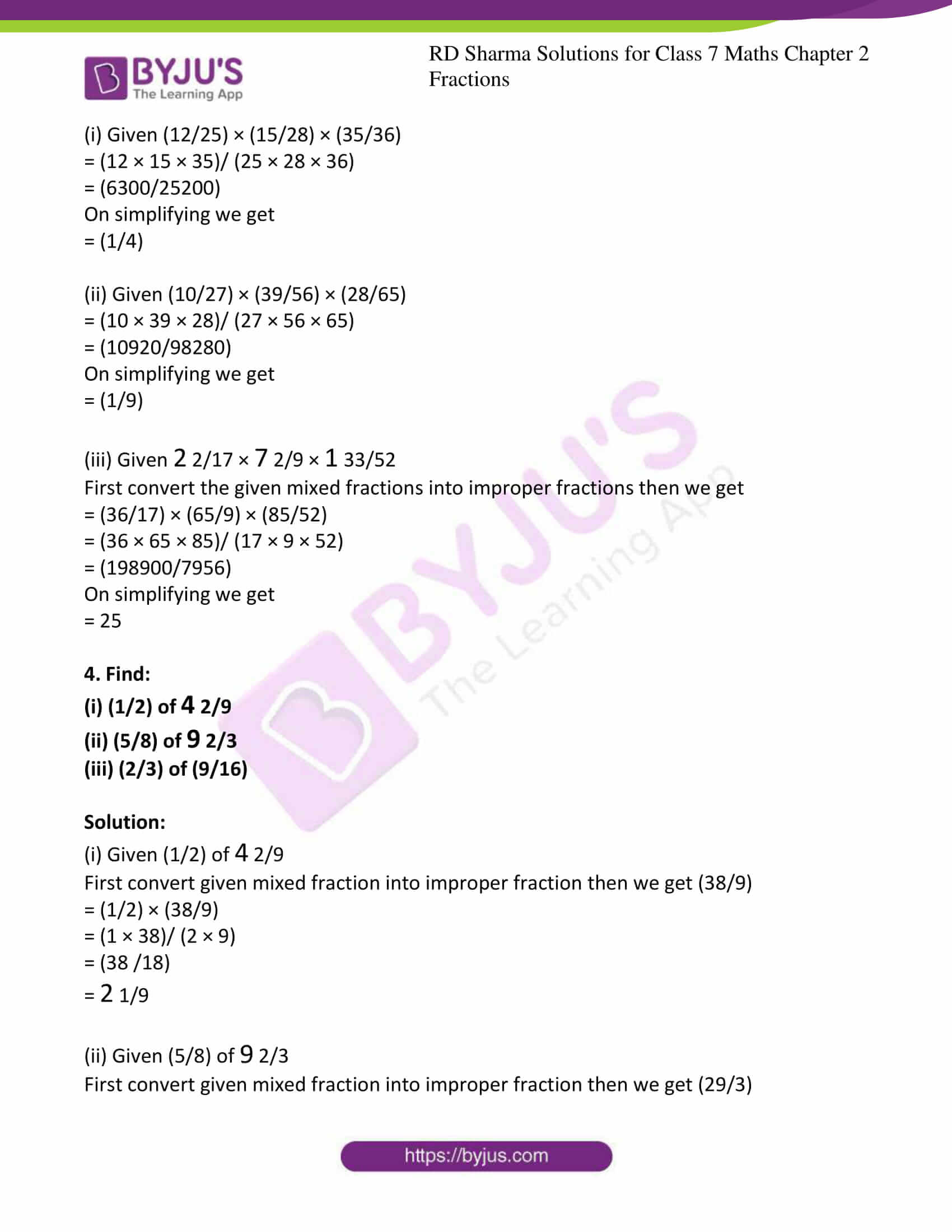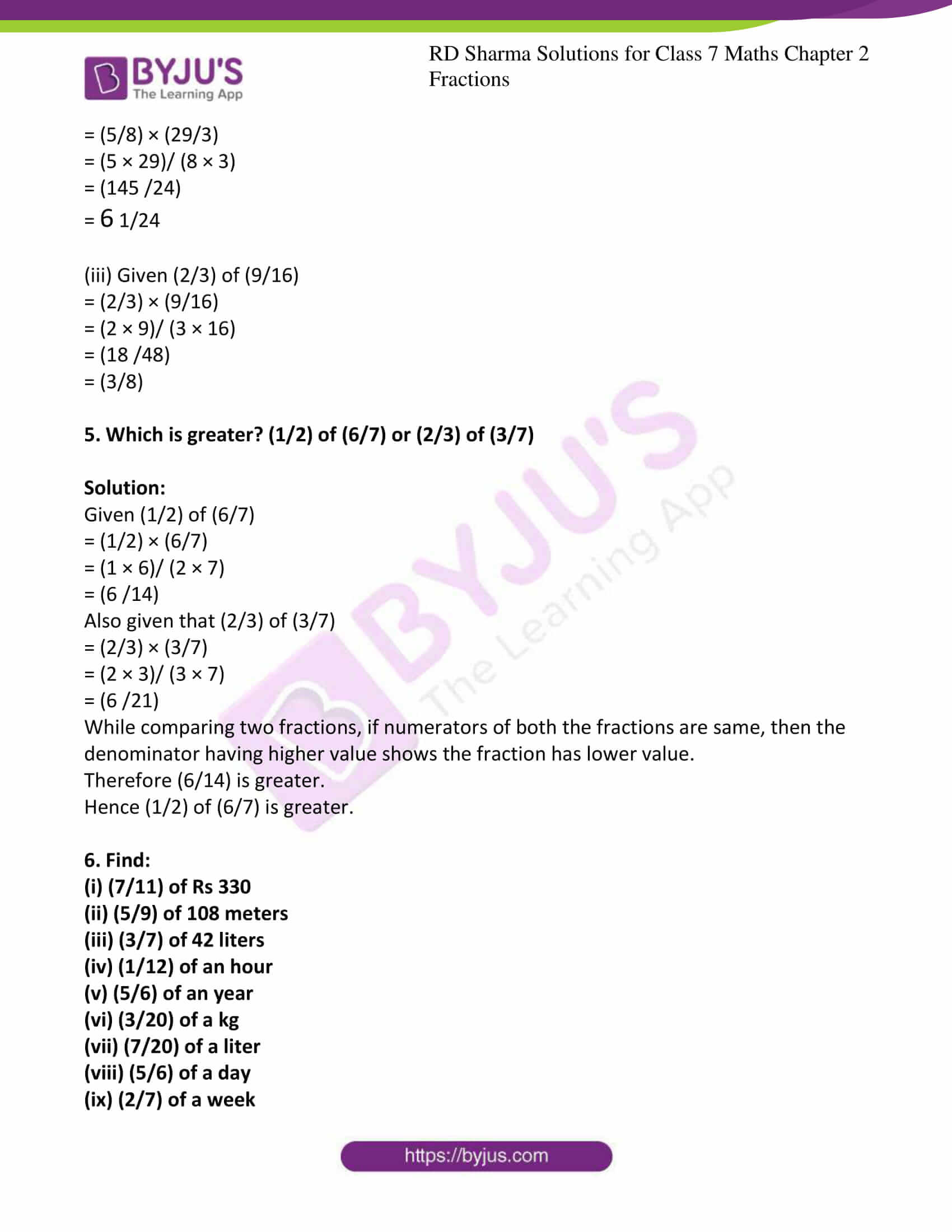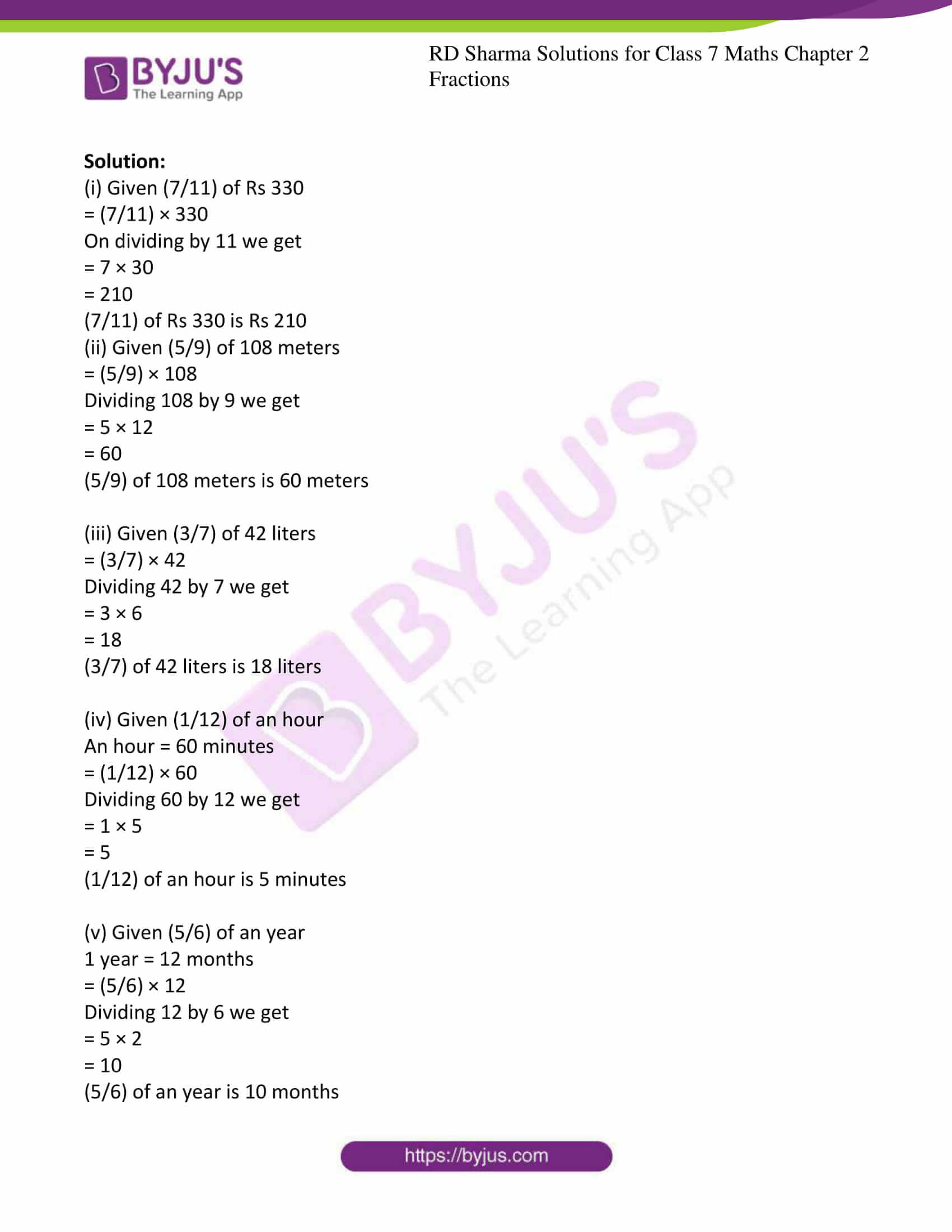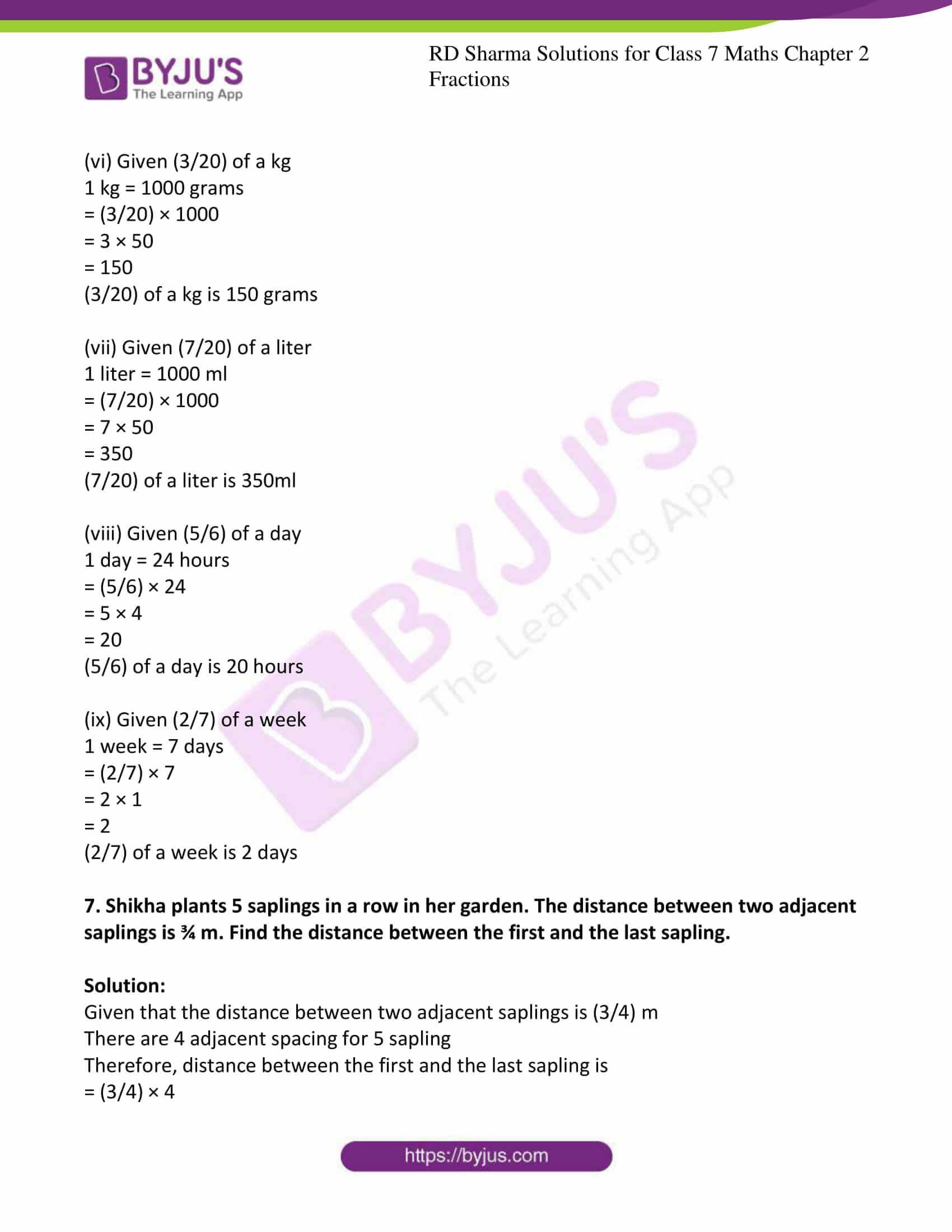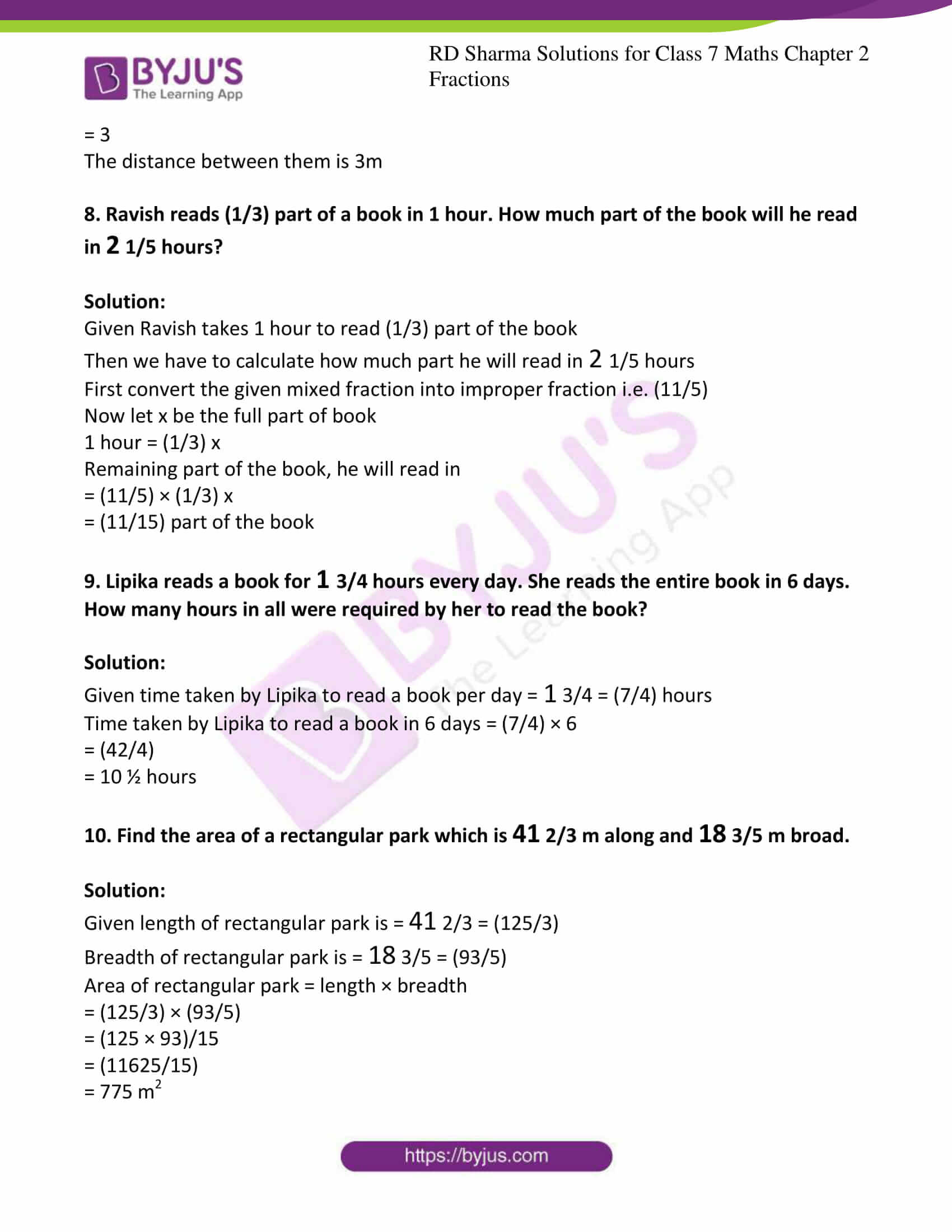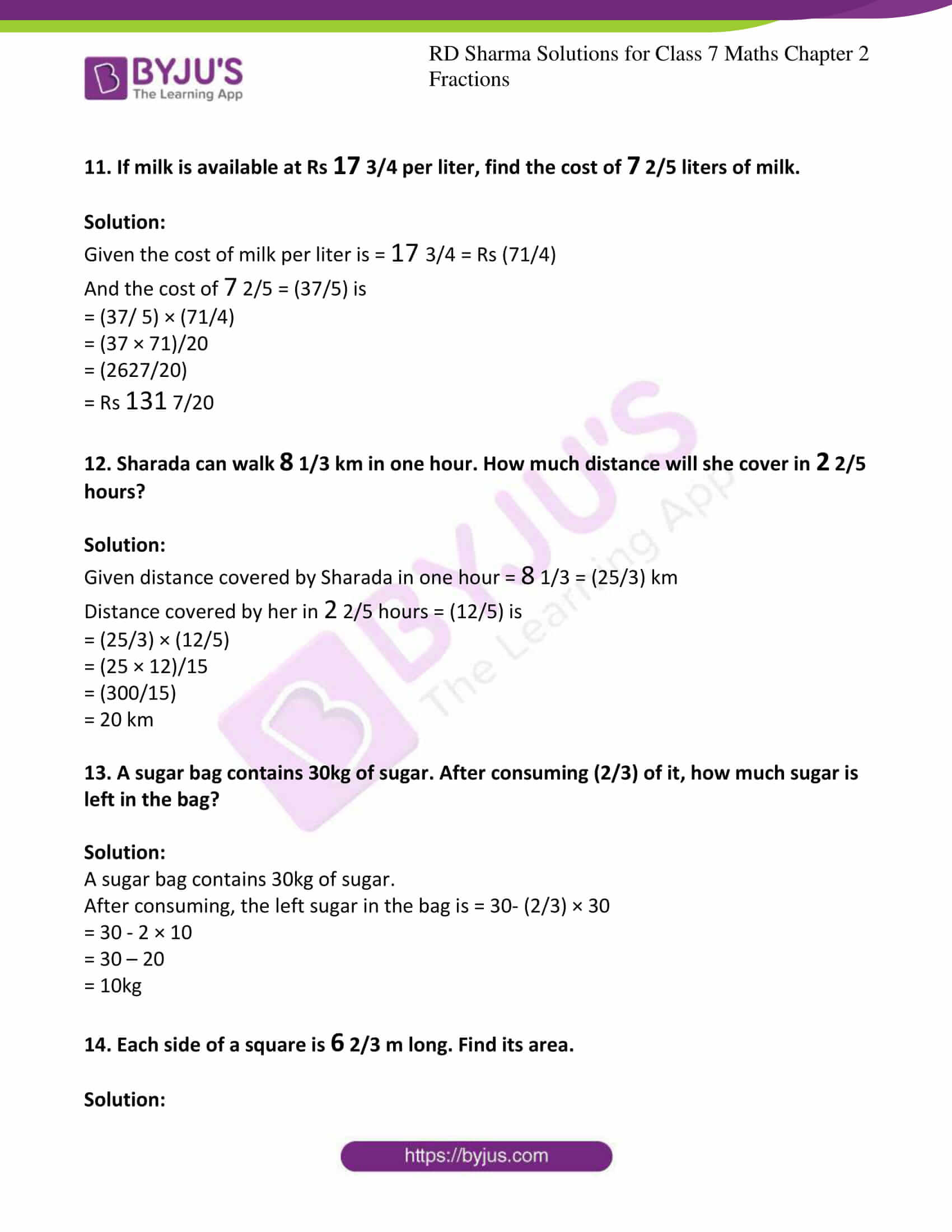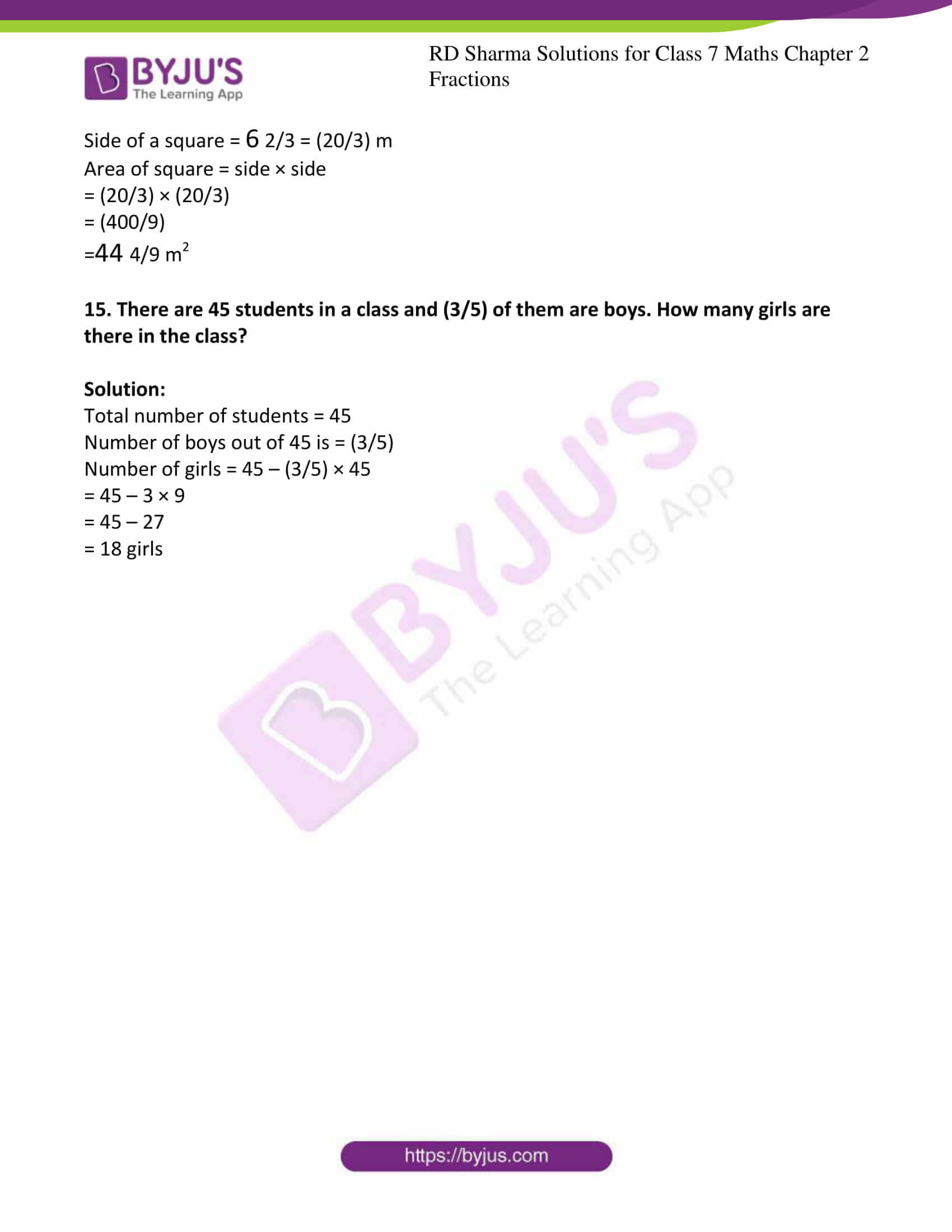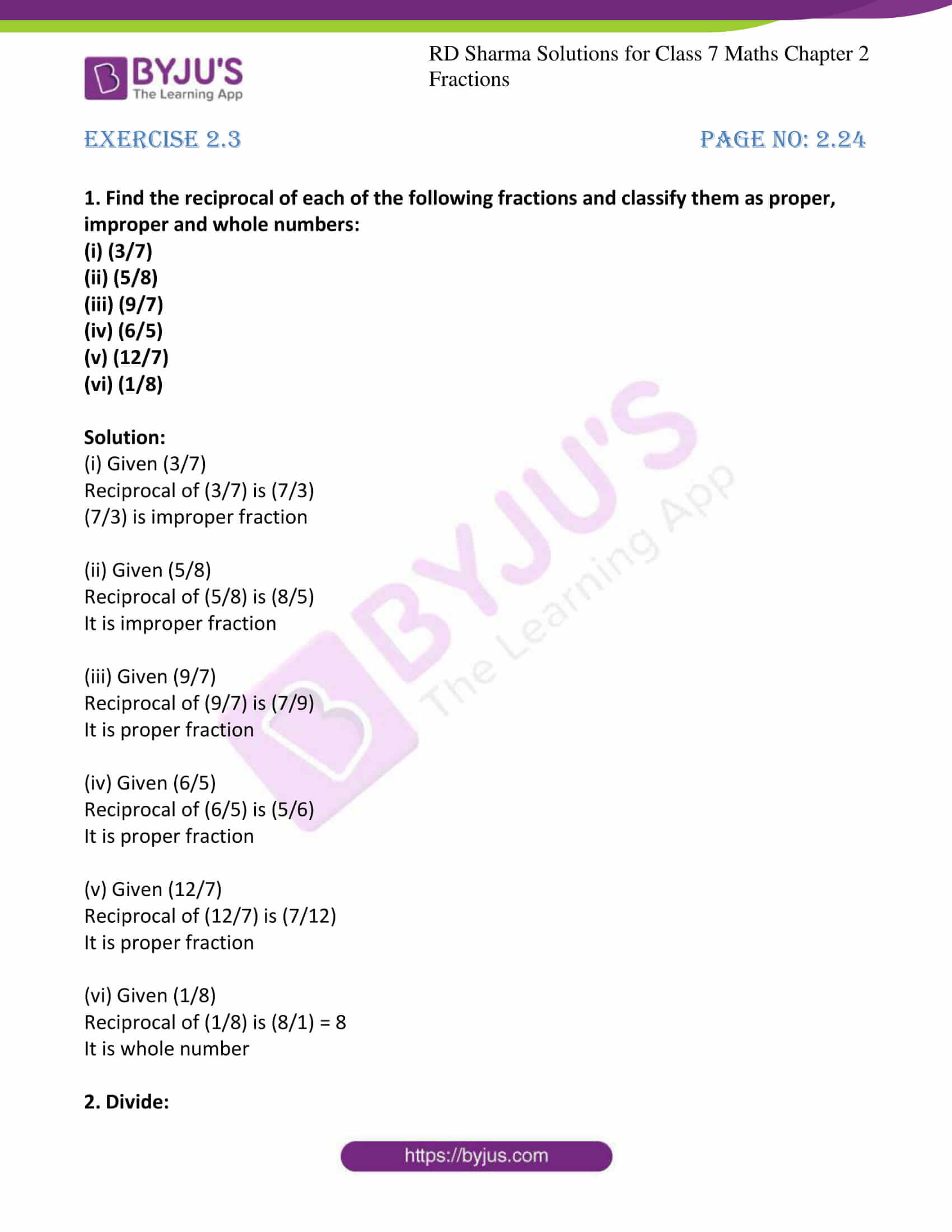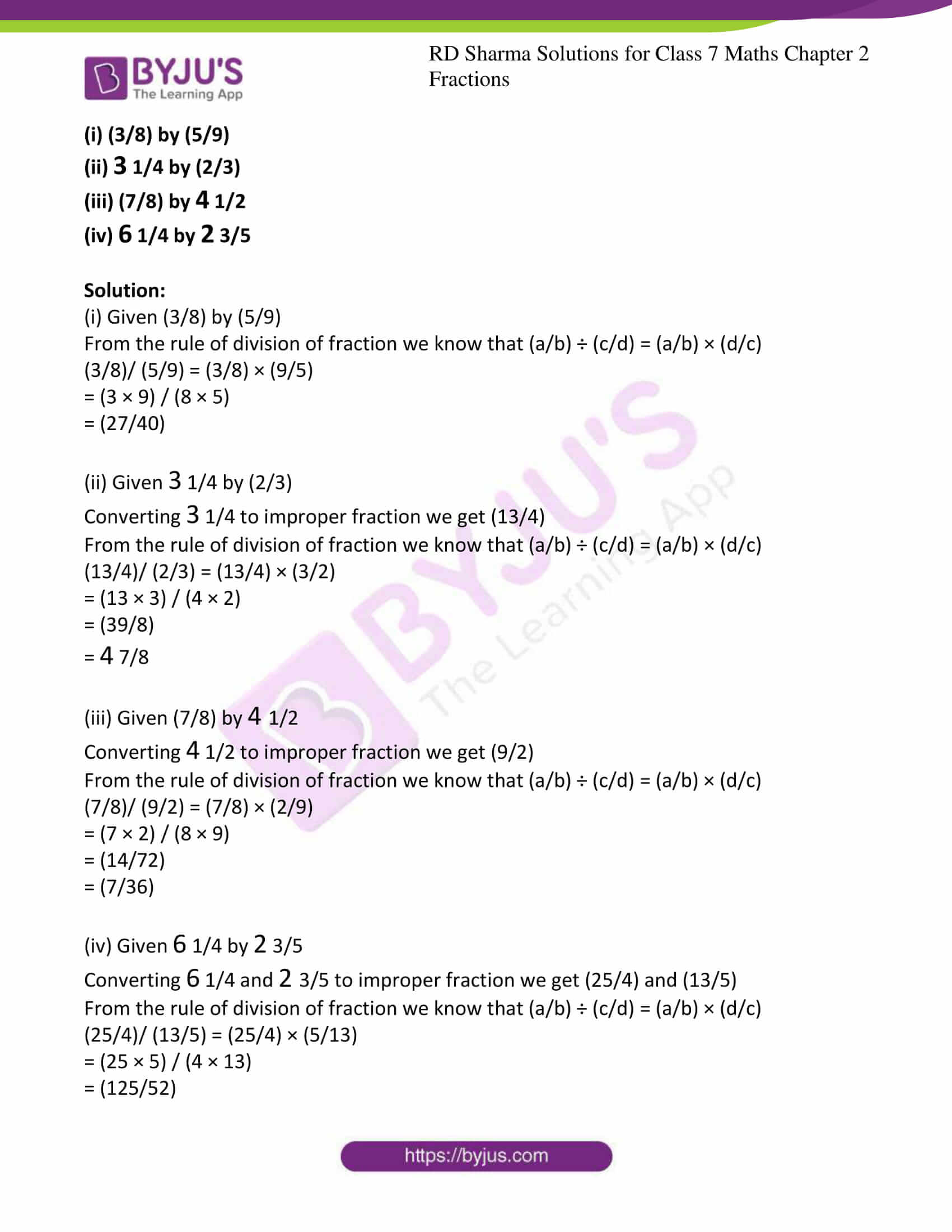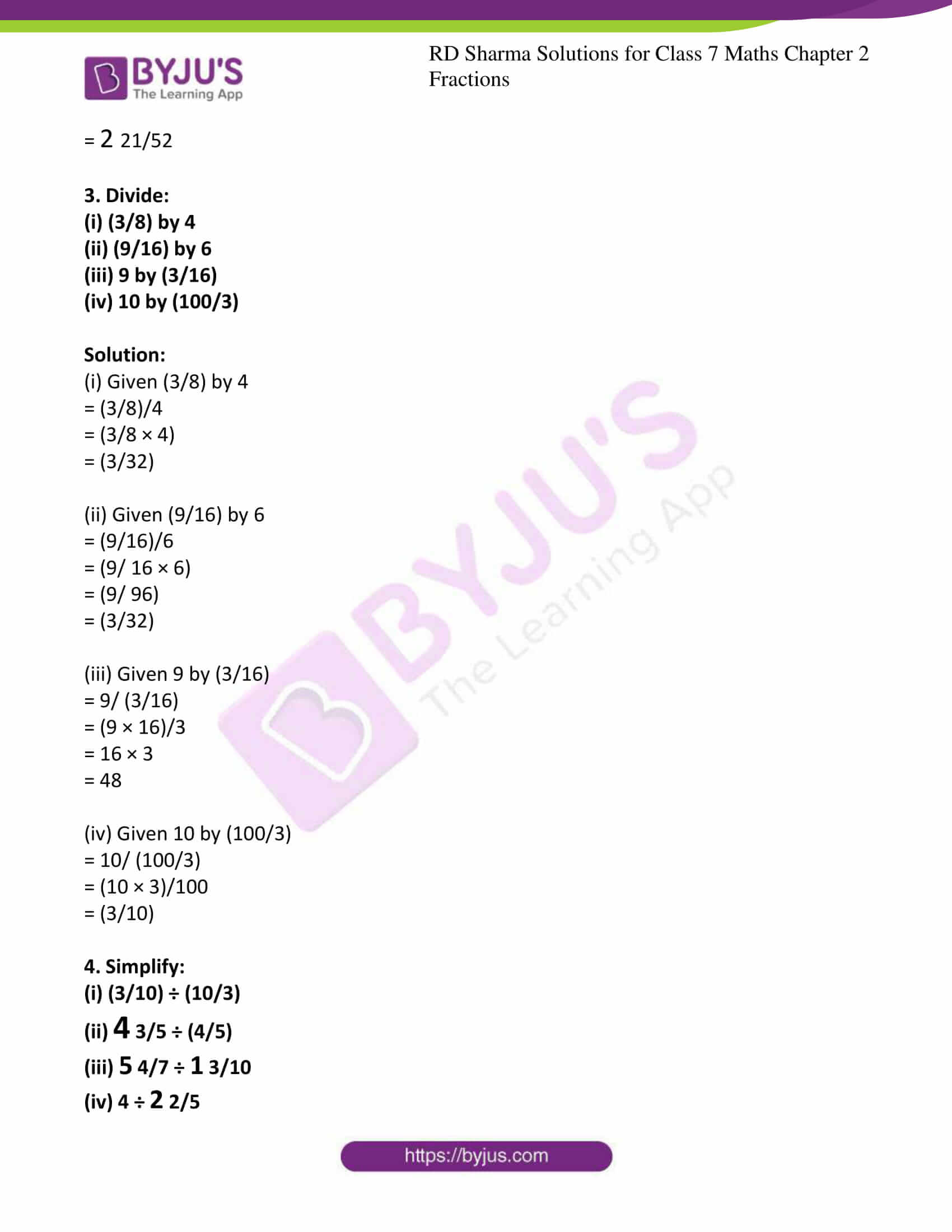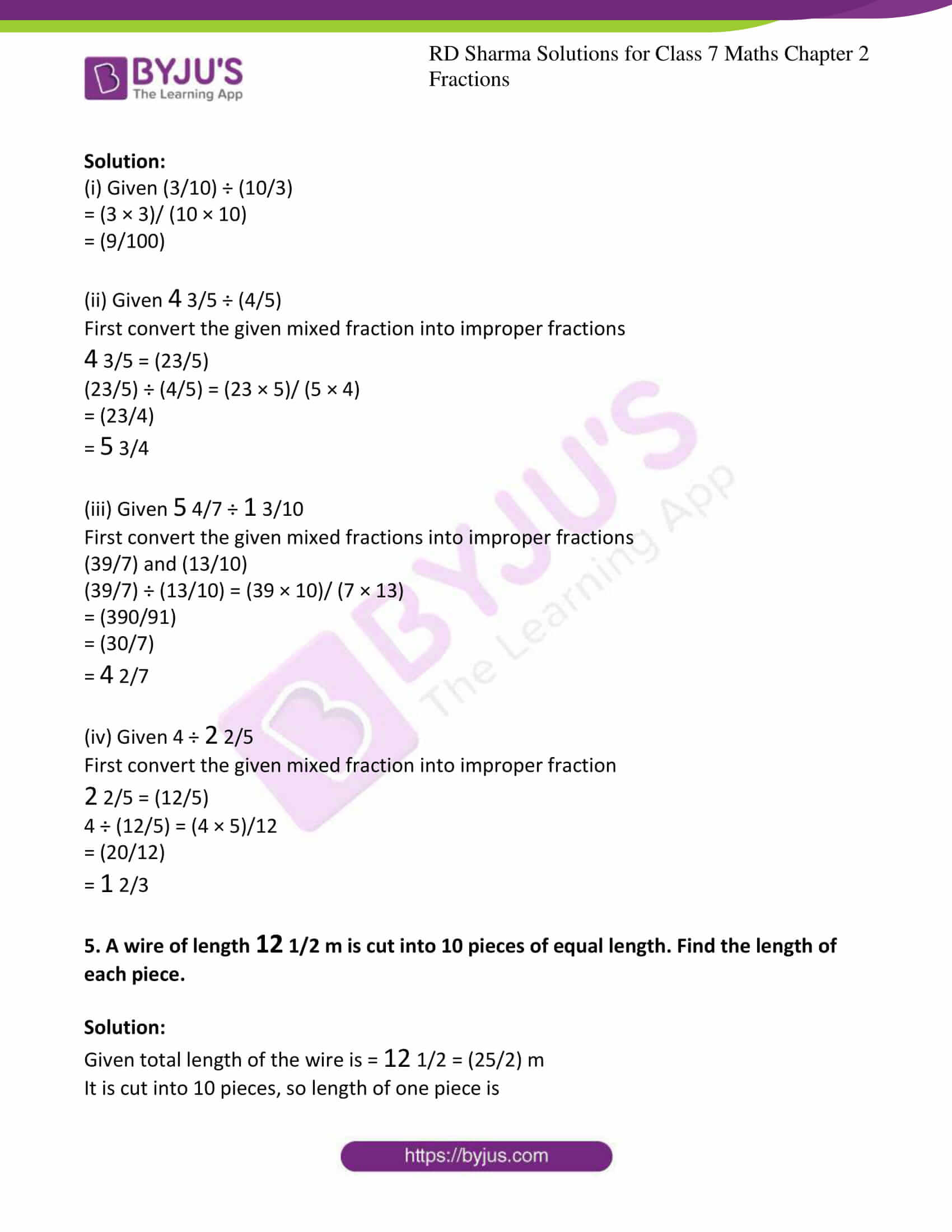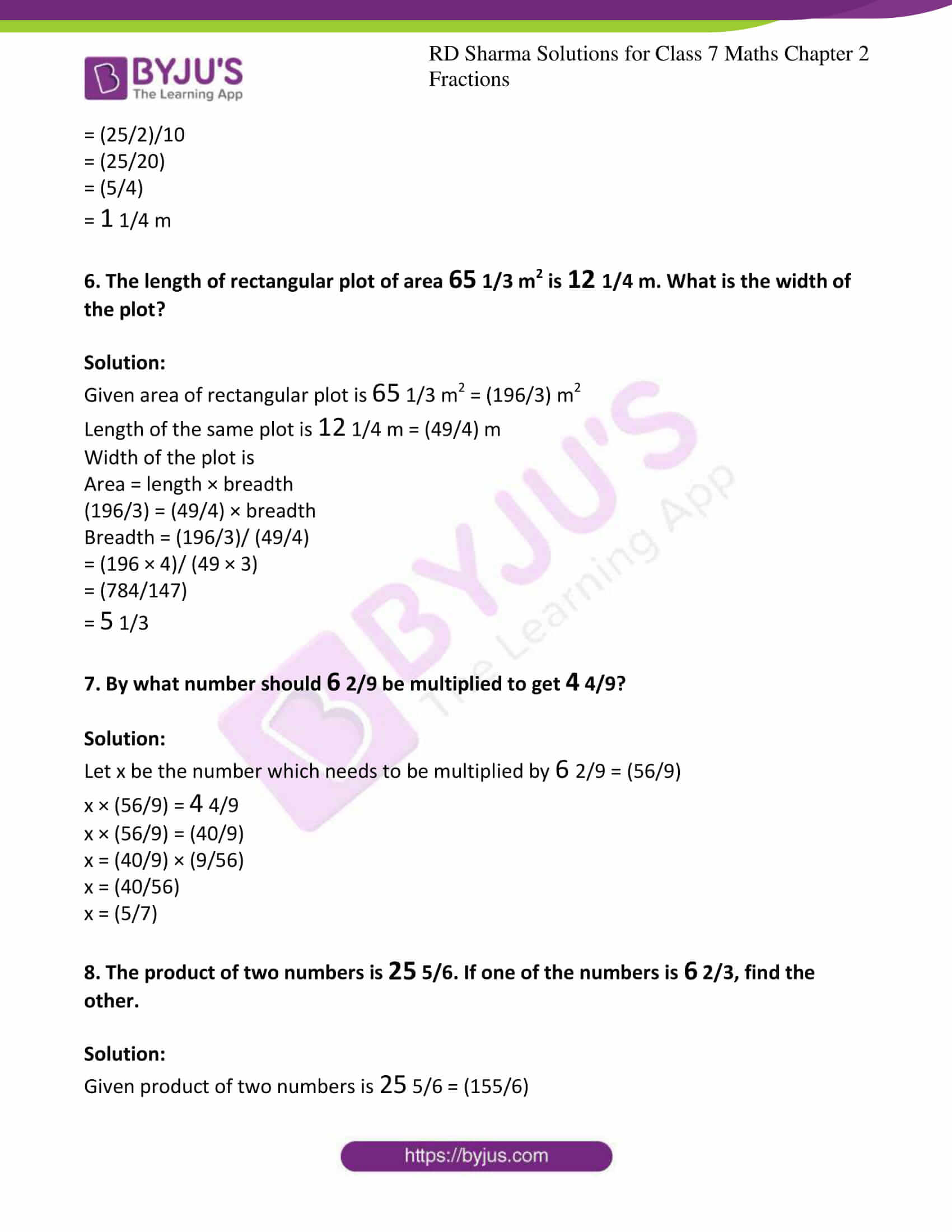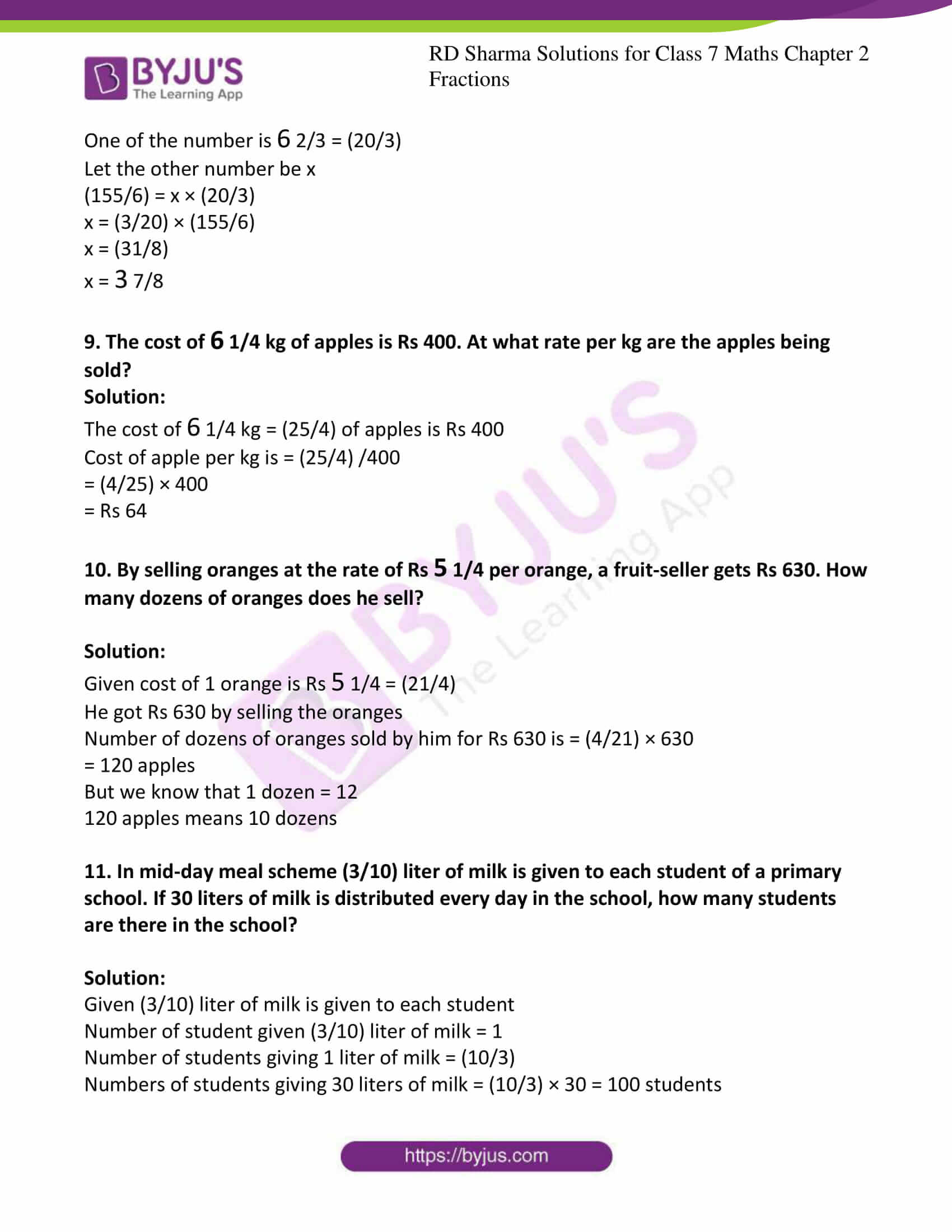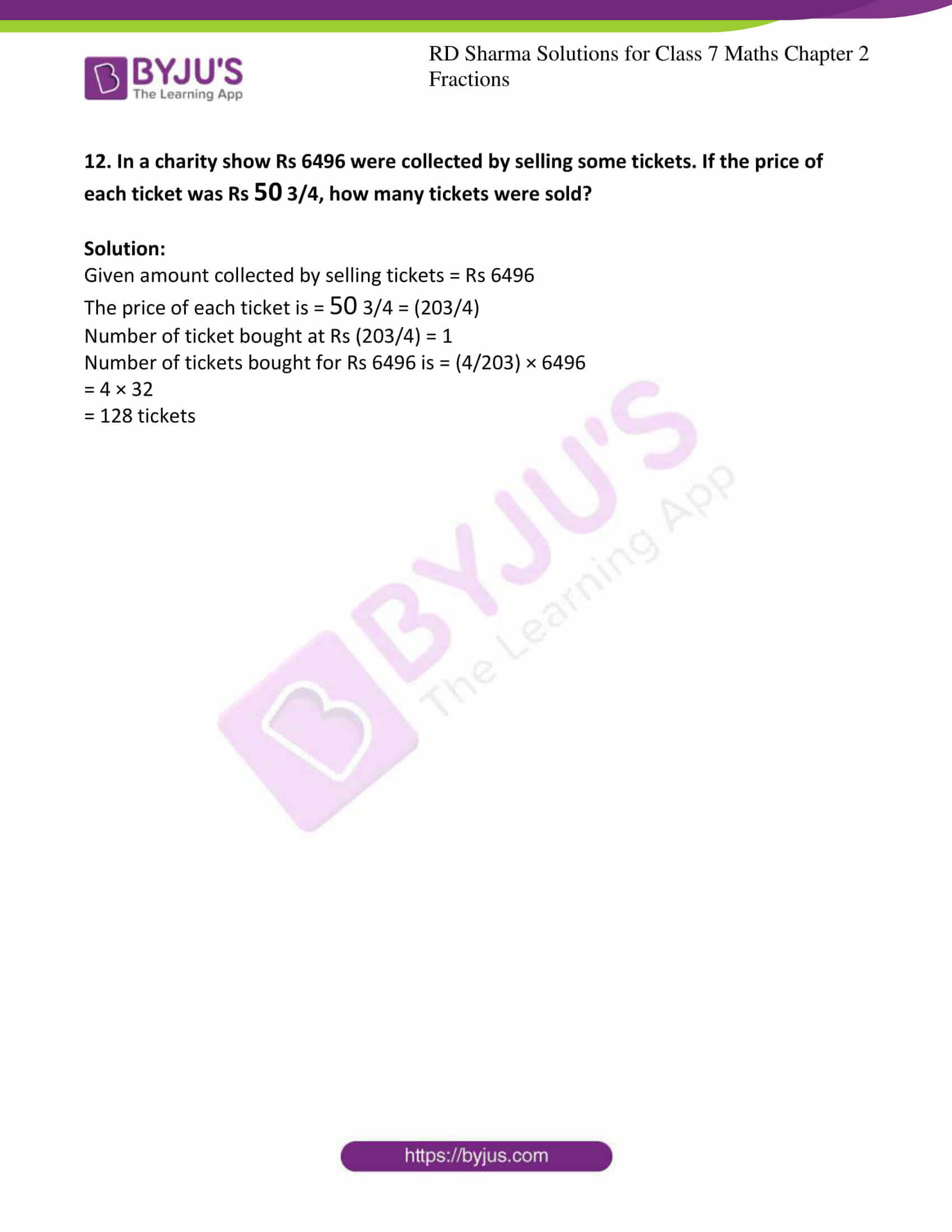### Access answers to Maths RD Sharma Solutions For Class 7 Chapter 2 – Fractions

Exercise 2.1 Page No: 2.12

1. Compare the following fractions by using the symbol > or < or =:

(i) (7/9) and (8/13)

(ii) (11/9) and (5/9)

(iii) (37/41) and (19/30)

(iv) (17/15) and (119/105)

Solution:

(i) Given (7/9) and (8/13)

Taking LCM for 9 and 13 we get,

9 × 13 = 117

Now we convert the given fractions into its equivalent fractions, then it becomes

(7 × 13)/ (9 × 13) and (8 × 9)/ (13 × 9)

Therefore (91/117) > (72/117)

Hence (7/9) > (8/13)

(ii) Given (11/9) and (5/9)

As the denominator is equal, they form equivalent fractions.

But we know that 11 > 5

Hence (11/9) > (5/9)

(iii) Given (37/41) and (19/30)

Taking LCM for 41 and 30 we get,

30 × 41 = 1230

Now we convert the given fractions into its equivalent fractions, then it becomes

(37 × 30)/ (41 × 30) and (19 × 41)/ (30 × 41)

Therefore (1110/1230) > (779/1230)

Hence (37/41) > (19/30)

(iv) Given (17/15) and (119/105)

Taking LCM for 15 and 105 we get, 5 × 3 × 7 = 105

Now we convert the given fractions into its equivalent fractions, then it becomes

(17 × 7)/ (15 × 7) and (119/105)

Therefore (119/105) = (119/105)

Hence (17/15) = (119/105)

2. Arrange the following fractions in ascending order:

(i) (3/8), (5/6), (6/8), (2/4), (1/3)

(ii) (4/6), (3/8), (6/12), (5/16)

Solution:

(i) Given (3/8), (5/6), (6/8), (2/4), (1/3)

Now we have to arrange these in ascending order, to arrange these in ascending order we have to make those as equivalent fractions by taking LCM’s.

LCM of 8, 6, 4 and 3 is 24

Equivalent fractions are

(9/24), (20/24), (18/24), (12/24), (8/24)

We know that 8 < 9 < 12 < 18 < 20

Now arranging in ascending order

(8/24) < (9/24) < (12/24) < (18/24) < (20/24)

Hence (1/3) < (3/8) < (2/4) < (6/8) < (5/6)

(ii) Given (4/6), (3/8), (6/12), (5/16)

Now we have to arrange these in ascending order, to arrange these in ascending order we have to make those as equivalent fractions by taking LCM’s.

LCM of 8, 6, 12 and 16 is 48

Equivalent fractions are

(32/48), (15/48), (18/48), (15/48)

We know that 12 < 15 < 18 < 32

Now arranging in ascending order

(12/48) < (15/48) < (18/48) < (32/48)

(6/12) < (5/16) < (3/8) < (4/6)

3. Arrange the following fractions in descending order:

(i) (4/5), (7/10), (11/15), (17/20)

(ii) (2/7), (11/35), (9/14), (13/28)

Solution:

(i) Given (4/5), (7/10), (11/15), (17/20)

Now we have to arrange these in descending order, to arrange these in descending order we have to make those as equivalent fractions by taking LCM’s.

LCM of 5, 10, 15 and 20 is 60

Equivalent fractions are

(48/60), (42/60), (44/60), (51/60)

We know that 51 > 48 > 44 > 42

Now arranging in descending order

Hence (17/20) > (4/5) > (11/15) > (7/10)

(ii) Given (2/7), (11/35), (9/14), (13/28)

Now we have to arrange these in descending order, to arrange these in descending order we have to make those as equivalent fractions by taking LCM’s.

LCM of 7, 35, 14 and 28 is 140

Equivalent fractions are

(40/140), (44/140), (90/140), (65/140)

We know that 90 > 65 > 44 > 40

Now arranging in descending order

Hence (9/14) > (13/28) > (11/35) > (2/7)

4. Write five equivalent fraction of (3/5).

Solution:

Given (3/5)

By multiplying or dividing both the numerator and denominator so that it keeps the same value by this we can get the equivalent fractions.

(3 × 2)/ (5 × 2), (3 × 3)/ (5 × 3), (3 × 4)/ (5 × 4), (3 × 5)/ (5 × 5), (3 × 6)/ (5 × 6)

Equivalent fractions are

(6/10), (9/15), (12/20), (15/25), (18/30)

5. Find the sum:

(i) (5/8) + (3/10)

(ii) 4 3/4 + 9 2/5

(iii) (5/6) + 3 + (3/4)

(iv) 2 3/5 + 4 7/10 + 2 4/15

Solution:

(i) Given (5/8) + (3/10)

Taking LCM for 8 and 10 we get 40

Now we have to convert the given fractions into equivalent fractions with denominator 40

(5/8) + (3/10) = (5 × 5)/ (8 × 5) + (3 × 4)/ (10 × 4)

= (25/40) + (12/40)

= (37/40)

(ii) Given 4 3/4 + 9 2/5

First convert given mixed fractions into improper fractions.

4 3/4 + 9 2/5 = (19/4) + (47/5)

Taking LCM for 4 and 5 we get 20

Now we have to convert the given fractions into equivalent fractions with denominator 20

4 3/4 + 9 2/5 = (19/4) + (47/5) = (19 × 5)/ (4 × 5) + (47 × 4)/ (5 × 4)

= (95/20) + (188/20)

= (283/20)

(iii) Given (5/6) + 3 + (3/4)

Taking LCM for 6, 1 and 4 we get 12

Now we have to convert the given fractions into equivalent fractions with denominator 12

(5/6) + 3 + (3/4) = (5 × 2)/ (6 × 2) + (3 × 12)/ (1 × 12) + (3 × 3)/ (4 × 3)

= (10/12) + (36/12) + (9/12)

= (55/12)

(iv) Given 2 3/5 + 4 7/10 + 2 4/15

First convert given mixed fractions into improper fractions

2 3/5 + 4 7/10 + 2 4/15 = (13/5) + (47/10) + (34/15)

Taking LCM for 5, 10 and 15 we get 30

Now we have to convert the given fractions into equivalent fractions with denominator 30

2 3/5 + 4 7/10 + 2 4/15 = (13/5) + (47/10) + (34/15) = (13 × 6)/ (5 × 6) + (47 × 3)/ (10 × 3) + (34 × 2)/ (15 × 2)

= (78/30) + (141/30) + (68/30)

= (287/30)

6. Find the difference of:

(i) (13/24) and (7/16)

(ii) 6 and (23/3)

(iii) (21/25) and (18/20)

(iv) 3 3/10 and 2 7/15

Solution:

(i) Given (13/24) and (7/16)

To find the difference we have to make it equivalent fractions.

LCM of 24 and 16 is 48.

Now converting the given fractions into equivalent fractions with denominator 48.

(13/24) – (7/16) = (26/48) – (21/48)

= (26 – 21)/48

= (5/48)

(ii) Given 6 and (23/3)

To find the difference we have to make it equivalent fractions.

LCM of 3 and 1 is 3.

Now converting the given fractions into equivalent fractions with denominator 3.

(23/3) – 6 = (23/3) – (18/3)

= (23 – 18)/3

= (5/3)

(iii) Given (21/25) and (18/20)

To find the difference we have to make it equivalent fractions.

LCM of 25 and 20 is 100.

Now converting the given fractions into equivalent fractions with denominator 100.

(18/20) – (21/25) = (90/100) – (84/100)

= (90 – 84)/100

= 6/100

By converting it into its simplest form we get

= 3/50

(iv) Given 3 3/10 and 2 7/15

First convert given mixed fractions into improper fractions.

(33/10) and (37/15)

To find the difference we have to make it equivalent fractions.

LCM of 10 and 15 is 30.

Now converting the given fractions into equivalent fractions with denominator 30.

(33/10) – (37/15) = (99/30) – (74/30)

= (99 – 74)/30

= (25/30)

By converting it into simplest form we get

= (5/6)

7. Find the difference:

(i) (6/7) – (9/11)

(ii) 8 – (5/9)

(iii) 9 – 5 2/3

(iv) 4 3/10 – 1 2/15

Solution:

(i) Given (6/7) – (9/11)

To find the difference we have to make it equivalent fractions.

LCM of 7 and 11 is 77.

Now converting the given fractions into equivalent fractions with denominator 77.

Equivalent fractions are (66/77) and (63/77)

(6/7) – (9/11) = (66/77) – (63/77)

= (66 – 63)/77

= (3/77)

(ii) Given 8 – (5/9)

To find the difference we have to make it equivalent fractions.

LCM of 1 and 9 is 9.

Now converting the given fractions into equivalent fractions with denominator 9.

Equivalent fractions are (72/9) and (5/9)

8 – (5/9) = (72/9) – (5/9)

= (72 -5)/9

= (67/9)

(iii) Given 9 – 5 2/3

First convert the given mixed fractions into improper fractions.

We get 5 2/3 = (17/3)

To find the difference we have to make it equivalent fractions.

LCM of 1 and 3 is 3.

Now converting the given fractions into equivalent fractions with denominator 3.

Equivalent fractions are (27/3) and (17/3)

9 – 5 2/3 = (27/3) – (17/3)

= (10/3)

(iv) Given 4 3/10 – 1 2/15

First convert the given mixed fractions into improper fractions.

We get (43/10) and (17/15)

To find the difference we have to make it equivalent fractions.

LCM of 10 and 15 is 30.

Now converting the given fractions into equivalent fractions with denominator 30.

Equivalent fractions are (129/30) and (34/30)

4 3/10 – 1 2/15 = (43/10) – (17/15)

= (129/30) – (34/30)

= (129 – 34)/30

= (95/30)

= (19/6)

8. Simplify:

(i) (2/3) + (1/6) – (2/9)

(ii) 12 – 3 1/2

(iii) 7 5/6 – 4 3/8 + 2 7/12

Solution:

(i) Given (2/3) + (1/6) – (2/9)

LCM of 3, 6 and 9 is 18

Now we have to convert the given fraction into equivalent fraction with denominator 18

(2/3) + (1/6) – (2/9) = (12/18) + (3/18) – (4/18)

= (12 + 3 – 4)/18

= 11/18

(ii) Given 12 – 3 (1/2)

First convert the given mixed fraction into improper fraction we get (7/2)

LCM of 2 and 1 is 2

Now we have to convert the given fraction into equivalent fraction with denominator 2

12 – 3 1/2 = (24/2) – (7/2)

= (24 -7)/2

= (17/2)

(iii) Given 7 5/6 – 4 3/8 + 2 7/12

First convert the given mixed fraction into improper fraction we get (47/6), (35/8) and (31/12)

LCM of 12, 6 and 8 is 48

Now we have to convert the given fraction into equivalent fraction with denominator 48

7 5/6 – 4 3/8 + 2 7/12 = (47/6) – (35/8) + (31/12)

= (376/48) – (210/48) + (124/48)

= (376 – 210 + 124)/48

= (290/48)

= (145/24)

9. What should be added to 5 3/7 to get 12?

Solution:

Given 5 3/7

First convert the given mixed fraction into improper fraction we get (38/7)

Let x be the number added to (38/7) to get 12

Therefore x + (38/7) = 12

x = 12 – (38/7)

LCM for 7 and 1 is 7

x = (12 × 7 – 38)/7

x = (84 – 38)/7

x = (46/7)

Hence (46/7) is the number which is added to 5 3/7 to get 12.

10. What should be added to 5 4/15 to get 12 3/5?

Solution:

Given 5 4/15

First convert the given mixed fraction into improper fraction we get (79/15)

Let x be the number added to (79/15) to get (63/5)

Therefore x + (79/15) = (63/5)

x = (63/5) – (79/15)

LCM for 15 and 5 is 15

x = (63 × 3 – 79)/15

x = (189 – 79)/15

x = (110/15) = (22/3)

Hence (22/3) is the number which is added to 5 4/15 to get 12 3/5.

11. Suman studies for 5 2/3 hours daily. She devotes 2 4/5 hours of her time for Science and Mathematics. How much time does she devote for other subject?

Solution:

Given Suman studies for 5 2/3 hours i.e. (17/3) hours

And she devotes 2 4/5 hours i.e. (14/5) hours for Science and Mathematics.

Let x be the hours she devotes for other subjects.

(17/3) = x + (14/5)

x = (17/3) – (14/5)

LCM of 3 and 5 is 15

x = (17 × 5 – 14× 3)/15

x = (85 – 42)/15

x = (43/15) = 2 13/15 hours

12. A piece of wire is of length 12 3/4 m. If it is cut into two pieces in such a way that the length of one piece is 5 1/4 m, what is the length of other piece?

Solution:

Given the total length of piece of wire is 12 3/4 i.e. (51/4) m

Length of one piece of wire is 5 1/4 i.e. ((21/4) m

Let the length of other piece be ‘x’ m

(51/4) = x + (21/4)

x = (51/4) – (21/4)

x = (51-21)/4

x = (30/4)

x = (15/2)

x = 7 1/2 m

13. A rectangular sheet of paper is 12 1/2 cm long and 10 2/3 cm wide. Find its perimeter.

Solution:

Given length of rectangular sheet of paper is 12 1/2 i.e. (25/2)

Breadth of rectangular sheet of paper is 10 2/3 i.e. (32/3)

But we know that perimeter of rectangle = 2 (length + breadth)

Perimeter of rectangular sheet = 2 [(25/2) + (32/3)]

LCM of 2 and 3 is 6, by taking this and simplifying we get

Perimeter = 2[(25 × 3)/6 + (32 × 2)/6]

= 2[(75/6) + (64/6)]

= 2(139/6)

= (139/3)

= 46 1/3 cm

14. In a “magic square”, the sum of the numbers in each row, in each column and along the diagonal is the same. Is this a magic square?

 (4/11) (9/11) (2/11) (3/11) (5/11) (7/11) (8/11) (1/11) (6/11)

Solution:

Along first column = (4/11) + (3/11) + (8/11) = (15/11)

Along second column = (9/11) + (5/11) + (7/11) = (15/11)

Along third column = (2/11) + (7/11) + (6/11) = (15/11)

Along first row = (4/11) + (9/11) + (2/11) = (15/11)

Along second row = (3/11) + (5/11) + (7/11) = (15/11)

Along third row = (8/11) + (1/11) + (6/11) = (15/11)

Along diagonal = (4/11) + (5/11) + (6/11) = (15/11)

= (2/11) + (5/11) + (8/11) = (15/11)

Therefore sum along all direction is same i.e. (15/11). Hence it is a magical square

15. The cost of Mathematics book is Rs 25 3/4 and that of Science book is Rs 20 1/2. Which cost more and by how much?

Solution:

Given the cost of Mathematics book is Rs 25 3/4 i.e. (103/4)

Cost of Science book is Rs 20 1/2 i.e. (41/2)

Now the LCM of 2 and 4 is 4

Now we have to convert the given fractions into its equivalent fractions with denominator 4

Mathematics book cost is Rs (103/4)

Science book cost is Rs (41 × 2/2 × 2) = (82/4)

(103 – 82)/4 = 21/4 = 5 1/4

Hence the cost of Mathematics book is more than cost of Science book by 5 1/4

16. (i) Provide the number in box [ ]and also give its simplest form in each of the following:

(i) (2/3) × [ ] = (10/30)

(ii) (3/5) × [ ] = (24/75)

Solution:

(i) (2/3) × [5/10] = (10/30)

(ii) (3/5) × [8/15] = (24/75)

Exercise 2.2 Page No: 2.19

1. Multiply:

(i) (7/11) by (3/5)

(ii) (3/5) by 25

(iii) 3 4/15 by 24

(iv) 3 1/8 by 4 10/11

Solution:

(i) Given (7/11) by (3/5)

We have to multiply the given number

(7/11) × (3/5) = (21/55)

(ii) Given (3/5) by 25

(3/5) × 25 = 15 [dividing 25 by 5]

(iii) Given 3 4/15 by 24

First convert the given mixed fraction to improper fraction.

(49/15) × 24 = (1176/15)

= 78 2/5

(iv) Given 3 1/8 by 4 10/11

First convert the given mixed fractions to improper fractions.

(25/8) × (54/11) = (1350/88) = (675/44)

= 15 15/44

2. Find the product:

(i) (4/7) × (14/25)

(ii) 7 1/2 × 2 4/15

(iii) 3 6/7 × 4 2/3

(iv) 6 11/14 × 3 1/2

Solution:

(i) Given (4/7) × (14/25)

(4/7) × (14/25) = (4 × 14)/ (7 × 25)

= (56/175)

Converting above fractions into simplest form

= (8/25)

(ii) Given 7 1/2 × 2 4/15

We have to convert mixed fractions into improper fractions

Then we get (15/2) and (34/15)

7 (1/2) × 2 (4/15) = (15/2) × (34/15)

= (15 × 34)/ (2 × 15)

= (510/30)

= 17

(iii) Given 3 6/7 × 4 2/3

We have to convert mixed fractions into improper fractions

Then we get (27/7) and (14/3)

3 6/7 × 4 2/3 = (27/7) × (14/3)

On simplifying

= 9 × 2

= 18

(iv) Given 6 11/14 × 3 1/2

We have to convert mixed fractions into improper fractions

Then we get (95/14) and (7/2)

6 11/14 × 3 1/2 = (95/14) × (7/2)

= (95 × 7)/28

= (665/28)

= 23 3/4

3. Simplify:

(i) (12/25) × (15/28) × (35/36)

(ii) (10/27) × (39/56) × (28/65)

(iii) 2 2/17 × 7 2/9 × 1 33/52

Solution:

(i) Given (12/25) × (15/28) × (35/36)

= (12 × 15 × 35)/ (25 × 28 × 36)

= (6300/25200)

On simplifying we get

= (1/4)

(ii) Given (10/27) × (39/56) × (28/65)

= (10 × 39 × 28)/ (27 × 56 × 65)

= (10920/98280)

On simplifying we get

= (1/9)

(iii) Given 2 2/17 × 7 2/9 × 1 33/52

First convert the given mixed fractions into improper fractions then we get

= (36/17) × (65/9) × (85/52)

= (36 × 65 × 85)/ (17 × 9 × 52)

= (198900/7956)

On simplifying we get

= 25

4. Find:

(i) (1/2) of 4 2/9

(ii) (5/8) of 9 2/3

(iii) (2/3) of (9/16)

Solution:

(i) Given (1/2) of 4 2/9

First convert given mixed fraction into improper fraction then we get (38/9)

= (1/2) × (38/9)

= (1 × 38)/ (2 × 9)

= (38 /18)

= 2 1/9

(ii) Given (5/8) of 9 2/3

First convert given mixed fraction into improper fraction then we get (29/3)

= (5/8) × (29/3)

= (5 × 29)/ (8 × 3)

= (145 /24)

= 6 1/24

(iii) Given (2/3) of (9/16)

= (2/3) × (9/16)

= (2 × 9)/ (3 × 16)

= (18 /48)

= (3/8)

5. Which is greater? (1/2) of (6/7) or (2/3) of (3/7)

Solution:

Given (1/2) of (6/7)

= (1/2) × (6/7)

= (1 × 6)/ (2 × 7)

= (6 /14)

Also given that (2/3) of (3/7)

= (2/3) × (3/7)

= (2 × 3)/ (3 × 7)

= (6 /21)

While comparing two fractions, if numerators of both the fractions are same, then the denominator having higher value shows the fraction has lower value.

Therefore (6/14) is greater.

Hence (1/2) of (6/7) is greater.

6. Find:

(i) (7/11) of Rs 330

(ii) (5/9) of 108 meters

(iii) (3/7) of 42 liters

(iv) (1/12) of an hour

(v) (5/6) of an year

(vi) (3/20) of a kg

(vii) (7/20) of a liter

(viii) (5/6) of a day

(ix) (2/7) of a week

Solution:

(i) Given (7/11) of Rs 330

= (7/11) × 330

On dividing by 11 we get

= 7 × 30

= 210

(7/11) of Rs 330 is Rs 210

(ii) Given (5/9) of 108 meters

= (5/9) × 108

Dividing 108 by 9 we get

= 5 × 12

= 60

(5/9) of 108 meters is 60 meters

(iii) Given (3/7) of 42 liters

= (3/7) × 42

Dividing 42 by 7 we get

= 3 × 6

= 18

(3/7) of 42 liters is 18 liters

(iv) Given (1/12) of an hour

An hour = 60 minutes

= (1/12) × 60

Dividing 60 by 12 we get

= 1 × 5

= 5

(1/12) of an hour is 5 minutes

(v) Given (5/6) of an year

1 year = 12 months

= (5/6) × 12

Dividing 12 by 6 we get

= 5 × 2

= 10

(5/6) of an year is 10 months

(vi) Given (3/20) of a kg

1 kg = 1000 grams

= (3/20) × 1000

= 3 × 50

= 150

(3/20) of a kg is 150 grams

(vii) Given (7/20) of a liter

1 liter = 1000 ml

= (7/20) × 1000

= 7 × 50

= 350

(7/20) of a liter is 350ml

(viii) Given (5/6) of a day

1 day = 24 hours

= (5/6) × 24

= 5 × 4

= 20

(5/6) of a day is 20 hours

(ix) Given (2/7) of a week

1 week = 7 days

= (2/7) × 7

= 2 × 1

= 2

(2/7) of a week is 2 days

7. Shikha plants 5 saplings in a row in her garden. The distance between two adjacent saplings is ¾ m. Find the distance between the first and the last sapling.

Solution:

Given that the distance between two adjacent saplings is (3/4) m

There are 4 adjacent spacing for 5 sapling

Therefore, distance between the first and the last sapling is

= (3/4) × 4

= 3

The distance between them is 3m

8. Ravish reads (1/3) part of a book in 1 hour. How much part of the book will he read in 2 1/5 hours?

Solution:

Given Ravish takes 1 hour to read (1/3) part of the book

Then we have to calculate how much part he will read in 2 1/5 hours

First convert the given mixed fraction into improper fraction i.e. (11/5)

Now let x be the full part of book

1 hour = (1/3) x

Remaining part of the book, he will read in

= (11/5) × (1/3) x

= (11/15) part of the book

9. Lipika reads a book for 1 3/4 hours every day. She reads the entire book in 6 days. How many hours in all were required by her to read the book?

Solution:

Given time taken by Lipika to read a book per day = 1 3/4 = (7/4) hours

Time taken by Lipika to read a book in 6 days = (7/4) × 6

= (42/4)

= 10 ½ hours

10. Find the area of a rectangular park which is 41 2/3 m along and 18 3/5 m broad.

Solution:

Given length of rectangular park is = 41 2/3 = (125/3)

Breadth of rectangular park is = 18 3/5 = (93/5)

Area of rectangular park = length × breadth

= (125/3) × (93/5)

= (125 × 93)/15

= (11625/15)

= 775 m2

11. If milk is available at Rs 17 3/4 per liter, find the cost of 7 2/5 liters of milk.

Solution:

Given the cost of milk per liter is = 17 3/4 = Rs (71/4)

And the cost of 7 2/5 = (37/5) is

= (37/ 5) × (71/4)

= (37 × 71)/20

= (2627/20)

= Rs 131 7/20

12. Sharada can walk 8 1/3 km in one hour. How much distance will she cover in 2 2/5 hours?

Solution:

Given distance covered by Sharada in one hour = 8 1/3 = (25/3) km

Distance covered by her in 2 2/5 hours = (12/5) is

= (25/3) × (12/5)

= (25 × 12)/15

= (300/15)

= 20 km

13. A sugar bag contains 30kg of sugar. After consuming (2/3) of it, how much sugar is left in the bag?

Solution:

A sugar bag contains 30kg of sugar.

After consuming, the left sugar in the bag is = 30- (2/3) × 30

= 30 – 2 × 10

= 30 – 20

= 10kg

14. Each side of a square is 6 2/3 m long. Find its area.

Solution:

Side of a square = 6 2/3 = (20/3) m

Area of square = side × side

= (20/3) × (20/3)

= (400/9)

=44 4/9 m2

15. There are 45 students in a class and (3/5) of them are boys. How many girls are there in the class?

Solution:

Total number of students = 45

Number of boys out of 45 is = (3/5)

Number of girls = 45 – (3/5) × 45

= 45 – 3 × 9

= 45 – 27

= 18 girls

Exercise 2.3 Page No: 2.24

1. Find the reciprocal of each of the following fractions and classify them as proper, improper and whole numbers:

(i) (3/7)

(ii) (5/8)

(iii) (9/7)

(iv) (6/5)

(v) (12/7)

(vi) (1/8)

Solution:

(i) Given (3/7)

Reciprocal of (3/7) is (7/3)

(7/3) is improper fraction

(ii) Given (5/8)

Reciprocal of (5/8) is (8/5)

It is improper fraction

(iii) Given (9/7)

Reciprocal of (9/7) is (7/9)

It is proper fraction

(iv) Given (6/5)

Reciprocal of (6/5) is (5/6)

It is proper fraction

(v) Given (12/7)

Reciprocal of (12/7) is (7/12)

It is proper fraction

(vi) Given (1/8)

Reciprocal of (1/8) is (8/1) = 8

It is whole number

2. Divide:

(i) (3/8) by (5/9)

(ii) 3 1/4 by (2/3)

(iii) (7/8) by 4 1/2

(iv) 6 1/4 by 2 3/5

Solution:

(i) Given (3/8) by (5/9)

From the rule of division of fraction we know that (a/b) ÷ (c/d) = (a/b) × (d/c)

(3/8)/ (5/9) = (3/8) × (9/5)

= (3 × 9) / (8 × 5)

= (27/40)

(ii) Given 3 1/4 by (2/3)

Converting 3 1/4 to improper fraction we get (13/4)

From the rule of division of fraction we know that (a/b) ÷ (c/d) = (a/b) × (d/c)

(13/4)/ (2/3) = (13/4) × (3/2)

= (13 × 3) / (4 × 2)

= (39/8)

= 4 7/8

(iii) Given (7/8) by 4 1/2

Converting 4 1/2 to improper fraction we get (9/2)

From the rule of division of fraction we know that (a/b) ÷ (c/d) = (a/b) × (d/c)

(7/8)/ (9/2) = (7/8) × (2/9)

= (7 × 2) / (8 × 9)

= (14/72)

= (7/36)

(iv) Given 6 1/4 by 2 3/5

Converting 6 1/4 and 2 3/5 to improper fraction we get (25/4) and (13/5)

From the rule of division of fraction we know that (a/b) ÷ (c/d) = (a/b) × (d/c)

(25/4)/ (13/5) = (25/4) × (5/13)

= (25 × 5) / (4 × 13)

= (125/52)

= 2 21/52

3. Divide:

(i) (3/8) by 4

(ii) (9/16) by 6

(iii) 9 by (3/16)

(iv) 10 by (100/3)

Solution:

(i) Given (3/8) by 4

= (3/8)/4

= (3/8 × 4)

= (3/32)

(ii) Given (9/16) by 6

= (9/16)/6

= (9/ 16 × 6)

= (9/ 96)

= (3/32)

(iii) Given 9 by (3/16)

= 9/ (3/16)

= (9 × 16)/3

= 16 × 3

= 48

(iv) Given 10 by (100/3)

= 10/ (100/3)

= (10 × 3)/100

= (3/10)

4. Simplify:

(i) (3/10) ÷ (10/3)

(ii) 4 3/5 ÷ (4/5)

(iii) 5 4/7 ÷ 1 3/10

(iv) 4 ÷ 2 2/5

Solution:

(i) Given (3/10) ÷ (10/3)

= (3 × 3)/ (10 × 10)

= (9/100)

(ii) Given 4 3/5 ÷ (4/5)

First convert the given mixed fraction into improper fractions

4 3/5 = (23/5)

(23/5) ÷ (4/5) = (23 × 5)/ (5 × 4)

= (23/4)

= 5 3/4

(iii) Given 5 4/7 ÷ 1 3/10

First convert the given mixed fractions into improper fractions

(39/7) and (13/10)

(39/7) ÷ (13/10) = (39 × 10)/ (7 × 13)

= (390/91)

= (30/7)

= 4 2/7

(iv) Given 4 ÷ 2 2/5

First convert the given mixed fraction into improper fraction

2 2/5 = (12/5)

4 ÷ (12/5) = (4 × 5)/12

= (20/12)

= 1 2/3

5. A wire of length 12 1/2 m is cut into 10 pieces of equal length. Find the length of each piece.

Solution:

Given total length of the wire is = 12 1/2 = (25/2) m

It is cut into 10 pieces, so length of one piece is

= (25/2)/10

= (25/20)

= (5/4)

= 1 1/4 m

6. The length of rectangular plot of area 65 1/3 m2 is 12 1/4 m. What is the width of the plot?

Solution:

Given area of rectangular plot is 65 1/3 m2 = (196/3) m2

Length of the same plot is 12 1/4 m = (49/4) m

Width of the plot is

= (196 × 4)/ (49 × 3)

= (784/147)

= 5 1/3

7. By what number should 6 2/9 be multiplied to get 4 4/9?

Solution:

Let x be the number which needs to be multiplied by 6 2/9 = (56/9)

x × (56/9) = 4 4/9

x × (56/9) = (40/9)

x = (40/9) × (9/56)

x = (40/56)

x = (5/7)

8. The product of two numbers is 25 5/6. If one of the numbers is 6 2/3, find the other.

Solution:

Given product of two numbers is 25 5/6 = (155/6)

One of the number is 6 2/3 = (20/3)

Let the other number be x

(155/6) = x × (20/3)

x = (3/20) × (155/6)

x = (31/8)

x = 3 7/8

9. The cost of 6 1/4 kg of apples is Rs 400. At what rate per kg are the apples being sold?

Solution:

The cost of 6 1/4 kg = (25/4) of apples is Rs 400

Cost of apple per kg is = (25/4) /400

= (4/25) × 400

= Rs 64

10. By selling oranges at the rate of Rs 5 1/4 per orange, a fruit-seller gets Rs 630. How many dozens of oranges does he sell?

Solution:

Given cost of 1 orange is Rs 5 1/4 = (21/4)

He got Rs 630 by selling the oranges

Number of dozens of oranges sold by him for Rs 630 is = (4/21) × 630

= 120 apples

But we know that 1 dozen = 12

120 apples means 10 dozens

11. In mid-day meal scheme (3/10) liter of milk is given to each student of a primary school. If 30 liters of milk is distributed every day in the school, how many students are there in the school?

Solution:

Given (3/10) liter of milk is given to each student

Number of student given (3/10) liter of milk = 1

Number of students giving 1 liter of milk = (10/3)

Numbers of students giving 30 liters of milk = (10/3) × 30 = 100 students

12. In a charity show Rs 6496 were collected by selling some tickets. If the price of each ticket was Rs 50 3/4, how many tickets were sold?

Solution:

Given amount collected by selling tickets = Rs 6496

The price of each ticket is = 50 3/4 = (203/4)

Number of ticket bought at Rs (203/4) = 1

Number of tickets bought for Rs 6496 is = (4/203) × 6496

= 4 × 32

= 128 tickets

### Chapter Brief of RD Sharma Solutions for Class 7 Maths Chapter 2 – Fractions

Chapter 2, Fractions, contains 3 Exercises. RD Sharma Solutions given here contains answers to all the questions present in these exercises. Let us have a look at some of the concepts that are being discussed in this Chapter.

• Definition of fractions
• Types of fractions
• Comparing fractions
• Conversion of unlike fractions to like fractions
• Addition and subtraction of fractions
• Multiplication of fractions
• Division of fractions

The Chapter Fractions explains fundamental operations like addition, subtraction, multiplication and division of fractions as well as comparison of fractions. A fraction can be defined as “a number representing a part of a whole”. Here the students will be thorough about the types of fractions, basic operations on them and so on.